# Overview of constrained PARAFAC models

## Abstract

In this paper, we present an overview of constrained parallel factor (PARAFAC) models where the constraints model linear dependencies among columns of the factor matrices of the tensor decomposition or, alternatively, the pattern of interactions between different modes of the tensor which are captured by the equivalent core tensor. Some tensor prerequisites with a particular emphasis on mode combination using Kronecker products of canonical vectors that makes easier matricization operations, are first introduced. This Kronecker product‐based approach is also formulated in terms of an index notation, which provides an original and concise formalism for both matricizing tensors and writing tensor models. Then, after a brief reminder of PARAFAC and Tucker models, two families of constrained tensor models, the co‐called PARALIND/CONFAC and PARATUCK models, are described in a unified framework, for N th‐order tensors. New tensor models, called nested Tucker models and block PARALIND/CONFAC models, are also introduced. A link between PARATUCK models and constrained PARAFAC models is then established. Finally, new uniqueness properties of PARATUCK models are deduced from sufficient conditions for essential uniqueness of their associated constrained PARAFAC models.

## 1 Review

### 1.1 Introduction

Tensor calculus was introduced in differential geometry, at the end of the nineteenth century, and then tensor analysis was developed in the context of Einstein’s theory of general relativity, with the introduction of index notation, the so‐called Einstein summation convention, at the beginning of the twentieth century, which allows to simplify and shorten physics equations involving tensors. Index notation is also useful for simplifying multivariate statistical calculations, particularly those involving cumulant tensors. Generally speaking, tensors are used in physics and differential geometry for characterizing the properties of a physical system, representing fundamental laws of physics, and defining geometrical objects whose components are functions. When these functions are defined over a continuum of points of a mathematical space, the tensor forms what is called a tensor field, a generalization of vector field used to solve problems involving curved surfaces or spaces, as it is the case of curved space‐time in general relativity. From a mathematical point of view, two other approaches are possible for defining tensors, in terms of tensor products of vector spaces, or multilinear maps. Symmetric tensors can also be linked with homogeneous polynomials.

After the first tensor developments by mathematicians and physicists, the need of analyzing collections of data matrices that can be seen as three‐way data arrays gave rise to three‐way models for data analysis, with the pioneering works of Tucker in psychometrics, and Harshman in phonetics, who proposed what is now referred to as the Tucker and parallel factor (PARAFAC) decompositions, respectively. The PARAFAC decomposition was independently proposed by Carroll and Chang under the name canonical decomposition (CANDECOMP) and then called CANDECOMP/PARAFAC (CP) in. For a history of the development of multi‐way models in the context of data analysis, see. Since the 1990s, multi‐way analysis has known a growing success in chemistry and especially in chemometrics (see Bro’s thesis and the book by Smilde et al. for a description of various chemical applications of three‐way models, with a pedagogical presentation of these models and of various algorithms for estimating their parameters). At the same period, tensor tools were developed for signal processing applications, more particularly for solving the so‐called blind source separation (BSS) problem using cumulant tensors (see‐ and De Lathauwer’s thesis where the concept of high‐order singular value decomposition (HOSVD) is introduced, a tensor tool generalizing the standard matrix SVD to arrays of order higher than two). A recent overview of BSS approaches and applications can be found in the handbook co‐edited by Comon and Jutten.

Nowadays, (high‐order) tensors, also called multi‐way arrays in the data analysis community, play an important role in many fields of application for representing and analyzing multidimensional data, as in psychometrics, chemometrics, food industry, environmental sciences, signal/image processing, computer vision, neuroscience, information sciences, data mining, pattern recognition, among many others. Then, they are simply considered as multidimensional arrays of numbers, constituting a generalization of vectors and matrices that are first‐ and second‐order tensors, respectively, to orders higher than two. Tensor decompositions, also called tensor models, are very useful for analyzing multidimensional data under the form of signals, images, speech, music sequences, or texts and also for designing new systems as it is the case of wireless communication systems since the publication of the seminal paper by Sidiropoulos et al.. Besides the references already cited, overviews of tensor tools, models, algorithms, and applications can be found in‐.

Tensor models incorporating constraints (sparsity; non‐negativity; smoothness; symmetry; column orthonormality of factor matrices; Hankel, Toeplitz, and Vandermonde structured matrix factors; allocation constraints...) have been the object of intensive works, during the last years. Such constraints can be inherent to the problem under study or the result of a system design. An overview of constraints on components of tensor models most often encountered in multi‐way data analysis can be found in. Incorporation of constraints in tensor models may facilitate physical interpretability of matrix factors. Moreover, imposing constraints may allow to relax uniqueness conditions and to develop specialized parameter estimation algorithms with improved performance both in terms of accuracy and computational cost, as it is the case of CP models with a column‐wise orthonormal factor matrix. One can classify the constraints into three main categories: i) sparsity/non‐negativity, ii) structural, and iii) linear dependencies/mode interactions. It is worth noting that the three categories of constraints involve specific parameter estimation algorithms, the first two generally inducing an improvement of uniqueness property of the tensor decomposition, while the third category implies a reduction of uniqueness, named partial uniqueness. We briefly review the main results concerning the first two types of constraints, Section 1.3 of this paper being dedicated to the third category.

Sparse and non‐negative tensor models have recently been the subject of many works in various fields of applications like computer vision[21, 22], image compression, hyperspectral imaging, music genre classification and audio source separation, multi‐channel EEG (electroencephalography) and network traffic analysis, fluorescence analysis, data denoising and image classification, among many others. Two non‐negative tensor models have been more particularly studied in the literature, the so‐called non‐negative tensor factorization (NTF), i.e., PARAFAC models with non‐negativity constraints on the matrix factors, and non‐negative Tucker decomposition (NTD), i.e., Tucker models with non‐negativity constraints on the core tensor and/or the matrix factors. The crucial importance of NTF/NTD for multi‐way data analysis applications results from the very large volume of real‐world data to be analyzed under constraints of sparseness and non‐negativity of factors to be estimated, when only non‐negative parameters are physically interpretable. Many NTF/NTD algorithms are now available. Most of them can be viewed as high‐order extensions of non‐negative matrix factorization (NMF) methods, in the sense that they are based on an alternating minimization of cost functions incorporating sparsity measures (also named distances or divergences) with application of NMF methods to matricized or vectorized forms of the tensor to be decomposed (see for instance[16, 23, 28, 30] for NTF and[29, 31] for NTD). An overview of NMF and NTF/NTD algorithms can be found in.

The second category of constraints concerns the case where the core tensor and/or some matrix factors of the tensor model have a special structure. For instance, we recently proposed a nonlinear CDMA scheme for multiuser SIMO communication systems that is based on a constrained block‐Tucker2 model whose core tensor, composed of the information symbols to be transmitted and their powers up to a certain degree, is characterized by matrix slices having a Vandermonde or a Hankel structure[32, 33]. We also developed Volterra‐PARAFAC models for nonlinear system modeling and identification. These models are obtained by expanding high‐order Volterra kernels, viewed as symmetric tensors, by means of symmetric or doubly symmetric PARAFAC decompositions[34, 35]. Block structured nonlinear systems like Wiener, Hammerstein, and parallel‐cascade Wiener systems can be identified from their associated Volterra kernels that admit symmetric PARAFAC decompositions with Toeplitz factors[36, 37]. Symmetric PARAFAC models with Hankel factors and symmetric block PARAFAC models with block Hankel factors are encountered for blind identification of multiple‐input multiple‐output (MIMO) linear channels using fourth‐order cumulant tensors, in the cases of memoryless and convolutive channels, respectively[38, 39]. In the presence of structural constraints, specific estimation algorithms can be derived as it is the case for symmetric CP decompositions, CP decompositions with Toeplitz factors (in, an iterative solution was proposed, whereas in, a non‐iterative algorithm was developed), Vandermonde factors, circulant factors, banded and/or structured matrix factors[45, 46], and also for Hankel and Vandermonde structured core tensors.

The rest of this paper is organized as follows: In Section 1.2, we present some tensor prerequisites with a particular emphasis on mode combination using Kronecker products of canonical vectors that makes easier the matricization operations, especially to derive matrix representations of tensor models. This Kronecker product‐based approach is also formulated in terms of an index notation, which provides an original and concise formalism for both matricizing tensors and writing tensor models. Then, we present the two most common tensor models, the so‐called Tucker and PARAFAC models, in a general framework, i.e., for N th‐order tensors. In Section 1.3, two families of constrained tensor models, the co‐called PARALIND/CONFAC and PARATUCK models, are described in a unified way, with a generalization to N th‐order tensors. New tensor models, called nested Tucker models and block PARALIND/CONFAC models, are also introduced. A link between PARATUCK models and constrained PARAFAC models is also established. In Section 1.4, uniqueness properties of PARATUCK models are deduced using this link. The paper is concluded in Section 2.

Notations and definitions.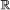and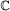denote the fields of real and complex numbers, respectively. Scalars, column vectors, matrices, and high‐order tensors are denoted by lowercase, boldface lowercase, boldface uppercase, and calligraphic uppercase letters, e.g., a, a, A, and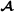, respectively. The vector Ai. (resp. A.j) represents the i th row (resp. j th column) of A.

I N ,${\mathbf{1}}_{N}^{T}$, and${\mathbf{e}}_{n}^{\left(N\right)}$ stand for the identity matrix of order N, the all‐ones row vector of dimensions 1×N, and the n th canonical vector of the Euclidean space${\mathbb{R}}^{N}$, respectively.

AT, AH, A, tr (A), and r A denote the transpose, the conjugate (Hermitian) transpose, the Moore‐Penrose pseudo‐inverse, the trace, and the rank of A, respectively. D i (A)=diag(Ai.) represents the diagonal matrix having the elements of the i th row of A on its diagonal. The operator bdiag(.) forms a block diagonal matrix from its matrix arguments, while the operator vec(.) transforms a matrix into a column vector by stacking the columns of its matrix argument one on top of the other one. In case of a tensor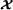, the vec(.) operation is defined in (6).

The outer product (also called tensor product), and the matrix Kronecker, Khatri‐Rao (column‐wise Kronecker), and Hadamard (element‐wise) products are denoted by , , , and , respectively.

Let us consider the set$\mathbb{S}=\left\{{n}_{1},\dots ,{n}_{N}\right\}$ obtained by permuting the elements of the set {1,…,N}. For${\mathbf{A}}^{\left(n\right)}\in {\mathbb{C}}^{{I}_{n}×{R}_{n}}$ and${\mathbf{u}}^{\left(n\right)}\in {\mathbb{C}}^{{I}_{n}×1}$, n=1,…,N, we define

$\begin{array}{ll}\underset{n\in \mathbb{S}}{\otimes }{\mathbf{A}}^{\left(n\right)}& ={\mathbf{A}}^{\left({n}_{1}\right)}\phantom{\rule{0.3em}{0ex}}\otimes \phantom{\rule{0.3em}{0ex}}{\mathbf{A}}^{\left({n}_{2}\right)}\phantom{\rule{0.3em}{0ex}}\otimes \cdots \otimes \phantom{\rule{0.3em}{0ex}}{\mathbf{A}}^{\left({n}_{N}\right)}\in \phantom{\rule{0.3em}{0ex}}{\mathbb{C}}^{{I}_{{n}_{1}}\cdots {I}_{{n}_{N}}×{R}_{{n}_{1}}\cdots {R}_{{n}_{N}}};\phantom{\rule{2em}{0ex}}\end{array}$
(1)
$\begin{array}{ll}\underset{n\in \mathbb{S}}{◇}{\mathbf{A}}^{\left(n\right)}& ={\mathbf{A}}^{\left({n}_{1}\right)}◇{\mathbf{A}}^{\left({n}_{2}\right)}◇\cdots ◇{\mathbf{A}}^{\left({n}_{N}\right)}\in {\mathbb{C}}^{{I}_{{n}_{1}}\cdots {I}_{{n}_{N}}×R},\phantom{\rule{2em}{0ex}}\\ \text{when}\phantom{\rule{2.77626pt}{0ex}}{R}_{n}=R,\forall n=1,\dots ,N;\phantom{\rule{2em}{0ex}}\\ \underset{n\in \mathbb{S}}{\odot }{\mathbf{A}}^{\left(n\right)}& ={\mathbf{A}}^{\left({n}_{1}\right)}\odot {\mathbf{A}}^{\left({n}_{2}\right)}\odot \cdots \odot {\mathbf{A}}^{\left({n}_{N}\right)}\in {\mathbb{C}}^{I×R},\phantom{\rule{2em}{0ex}}\\ \text{when}\phantom{\rule{2.77626pt}{0ex}}{I}_{n}=I,\phantom{\rule{2.77626pt}{0ex}}\text{and}\phantom{\rule{2.77626pt}{0ex}}{R}_{n}=R,\phantom{\rule{0.3em}{0ex}}\forall n=1,\dots ,N;\phantom{\rule{2em}{0ex}}\\ \underset{n\in \mathbb{S}}{\circ }{\mathbf{u}}^{\left(n\right)}& ={\mathbf{u}}^{\left({n}_{1}\right)}\circ {\mathbf{u}}^{\left({n}_{2}\right)}\circ \cdots \circ {\mathbf{u}}^{\left({n}_{N}\right)}\in {\mathbb{C}}^{{I}_{{n}_{1}}×\cdots ×{I}_{{n}_{N}}}.\phantom{\rule{2em}{0ex}}\end{array}$
(2)

The outer product of N non‐zero vectors defines a rank‐one tensor of order N.

By convention, the order of dimensions is directly related to the order of variation of the associated indices. For instance, in (1) and (2), the product${I}_{{n}_{1}}{I}_{{n}_{2}}\cdots {I}_{{n}_{N}}$ of dimensions means that n1 is the index varying the most slowly while n N is the index varying the most fastly in the Kronecker products computation.

For$\mathbb{S}=\left\{1,\dots ,N\right\}$, we have the following identities:

$\begin{array}{ll}{\left(\underset{n\in \mathbb{S}}{\circ }{\mathbf{u}}^{\left(n\right)}\right)}_{{i}_{1},\dots ,{i}_{N}}& ={\left(\stackrel{N}{\underset{n=1}{\circ }}{\mathbf{u}}^{\left(n\right)}\right)}_{{i}_{1},\dots ,{i}_{N}}=\prod _{n=1}^{N}{u}_{{i}_{n}}^{\left(n\right)},\phantom{\rule{2em}{0ex}}\\ {\left(\underset{n\in \mathbb{S}}{\otimes }{\mathbf{u}}^{\left(n\right)}\right)}_{i}& ={\left(\stackrel{N}{\underset{n=1}{\otimes }}{\mathbf{u}}^{\left(n\right)}\right)}_{i}=\prod _{n=1}^{N}{u}_{{i}_{n}}^{\left(n\right)}\phantom{\rule{1em}{0ex}}\phantom{\rule{2em}{0ex}}\\ \text{with}\phantom{\rule{1em}{0ex}}i& ={i}_{N}+\sum _{n=1}^{N-1}\left({i}_{n}-1\right)\prod _{j=n+1}^{N}{I}_{j}.\phantom{\rule{2em}{0ex}}\end{array}$
(3)

In particular, for$\mathbf{u}\in {\mathbb{C}}^{I×1},\mathbf{v}\in {\mathbb{C}}^{\phantom{\rule{0.3em}{0ex}}J×1},\mathbf{w}\in {\mathbb{C}}^{K×1}$

$\begin{array}{ll}\phantom{\rule{-10.0pt}{0ex}}\mathcal{X}& =\mathbf{u}\circ \mathbf{v}\circ \mathbf{w}\in {\mathbb{C}}^{I×J×K}⇔{x}_{\mathit{\text{ijk}}}={u}_{i}{v}_{j}{w}_{k},\phantom{\rule{2em}{0ex}}\\ \phantom{\rule{-10.0pt}{0ex}}\mathbf{x}& =\mathbf{u}\otimes \mathbf{v}\otimes \mathbf{w}\in {\mathbb{C}}^{\mathit{\text{IJK}}×1}⇔{x}_{k+\left(\phantom{\rule{0.3em}{0ex}}j-1\right)K+\left(i-1\right)\phantom{\rule{0.3em}{0ex}}\mathit{\text{JK}}}={u}_{i}{v}_{j}{w}_{k}.\phantom{\rule{2em}{0ex}}\end{array}$

Some useful matrix formulae are recalled in Appendix 1.

### 1.2 Tensor prerequisites

In this paper, a tensor is simply viewed as a multidimensional array of measurements. Depending that these measurements are real‐ or complex‐valued, we have a real‐ or complex‐valued tensor, respectively. The order N of a tensor refers to the number of indices that characterize its elements${x}_{{i}_{1},\dots ,{i}_{N}}$, each index i n (i n =1,…,I N ,for n=1,…,N) being associated with a dimension, also called a way, or a mode, and I n denoting the mode‐n dimension.

An N th‐order complex‐valued tensor$\mathcal{X}\in {\mathbb{C}}^{{I}_{1}×\cdots ×{I}_{N}}$, also called an N‐way array, of dimensions I1××I N , can be written as

$\mathcal{X}=\sum _{{i}_{1}=1}^{{I}_{1}}\cdots \sum _{{i}_{N}=1}^{{I}_{N}}{x}_{{i}_{1},\dots ,{i}_{N}}\stackrel{N}{\underset{n=1}{\circ }}{\mathbf{e}}_{{i}_{n}}^{\left({I}_{n}\right)}.$
(4)

The coefficients${x}_{{i}_{1},\dots ,{i}_{N}}$ represent the coordinates ofin the canonical basis$\left\{\phantom{\rule{0.3em}{0ex}}\stackrel{N}{\underset{n=1}{\circ }}{\mathbf{e}}_{{i}_{n}}^{\left({I}_{n}\right)},{i}_{n}=1,\dots ,{I}_{n};n=1,\dots ,N\phantom{\rule{0.3em}{0ex}}\right\}$ of the space${\mathbb{C}}^{{I}_{1}×\cdots ×{I}_{N}}$.

The identity tensor of order N and dimensions I××I, denoted by${\mathcal{I}}_{N,I}$ or simply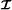, is a diagonal hypercubic tensor whose elements${\delta }_{{i}_{1},\dots ,{i}_{N}}$ are defined by means of the generalized Kronecker delta, i.e.,${\delta }_{{i}_{1},\dots ,{i}_{N}}=\left\{\begin{array}{c}1\phantom{\rule{1em}{0ex}}\text{if}\phantom{\rule{1em}{0ex}}{i}_{1}=\cdots ={i}_{N}\\ 0\phantom{\rule{1em}{0ex}}\text{otherwise}\end{array}\right\$, and I n =I,n=1,…,N. It can be written as

${\mathcal{I}}_{N,I}=\sum _{i=1}^{I}\underset{N\phantom{\rule{0.3em}{0ex}}\phantom{\rule{0.3em}{0ex}}\text{terms}}{\underset{⏟}{{\mathbf{e}}_{i}^{\left(I\right)}\circ \cdots \circ {\mathbf{e}}_{i}^{\left(I\right)}}}.$

Different reduced order tensors can be obtained by slicing the tensor$\mathcal{X}\in {\mathbb{C}}^{{I}_{1}×\cdots ×{I}_{N}}$ along one mode or p modes, i.e., by fixing one index i n or a set of p indices$\left\{{i}_{{n}_{1}},\dots ,{i}_{{n}_{p}}\right\}$, which gives a tensor of order N-1 or N-p, respectively. For instance, by slicingalong its mode‐n, we get the${i}_{n}^{\text{th}}$ mode‐n slice of, denoted by${\mathcal{X}}_{\dots {i}_{n}\dots }$, that can be written as

$\begin{array}{ll}{\mathcal{X}}_{\dots {i}_{n}\dots }& =\sum _{{i}_{1}=1}^{{I}_{1}}\cdots \sum _{{i}_{n-1}=1}^{{I}_{n-1}}\sum _{{i}_{n+1}=1}^{{I}_{n+1}}\cdots \sum _{{i}_{N}=1}^{{I}_{N}}{x}_{{i}_{1},\dots ,{i}_{n},\dots ,{i}_{N}}\phantom{\rule{2em}{0ex}}\\ \phantom{\rule{1em}{0ex}}×{\mathbf{e}}_{{i}_{n+1}}^{\left({I}_{n+1}\right)}\circ \cdots \circ {\mathbf{e}}_{{i}_{N}}^{\left({I}_{N}\right)}\circ {\mathbf{e}}_{{i}_{1}}^{\left({I}_{1}\right)}\circ \cdots \phantom{\rule{2em}{0ex}}\\ \phantom{\rule{1em}{0ex}}\cdots \circ {\mathbf{e}}_{{i}_{n-1}}^{\left({I}_{n-1}\right)}\in {\mathbb{C}}^{{I}_{n+1}×\cdots ×{I}_{N}×{I}_{1}×\cdots ×{I}_{n-1}}.\phantom{\rule{2em}{0ex}}\end{array}$

For instance, by slicing the third‐order tensor$\mathcal{X}\in {\mathbb{C}}^{I×J×K}$ along each mode, we get three types of matrix slices, respectively called horizontal, lateral, and frontal slices:

$\begin{array}{l}{\mathbf{X}}_{\mathrm{i..}}\in {\mathbb{C}}^{J×K},{\mathbf{X}}_{\mathrm{.j.}}\in {\mathbb{C}}^{K×I}\phantom{\rule{0.3em}{0ex}}\phantom{\rule{0.3em}{0ex}}\text{and}\phantom{\rule{0.3em}{0ex}}\phantom{\rule{0.3em}{0ex}}{\mathbf{X}}_{\mathrm{..k}}\in {\mathbb{C}}^{I×J},\phantom{\rule{2em}{0ex}}\\ \text{with}\phantom{\rule{0.3em}{0ex}}\phantom{\rule{0.3em}{0ex}}i=1,\dots ,I;j=1,\dots ,J;k=1,\dots ,\mathrm{K.}\phantom{\rule{2em}{0ex}}\end{array}$

Consider$\mathcal{A}\phantom{\rule{0.3em}{0ex}}\in \phantom{\rule{0.3em}{0ex}}{\mathbb{C}}^{{R}_{1}\phantom{\rule{0.3em}{0ex}}×\phantom{\rule{0.3em}{0ex}}\cdots \phantom{\rule{0.3em}{0ex}}×\phantom{\rule{0.3em}{0ex}}{R}_{N}\phantom{\rule{0.3em}{0ex}}×\phantom{\rule{0.3em}{0ex}}{I}_{1}\phantom{\rule{0.3em}{0ex}}×\phantom{\rule{0.3em}{0ex}}\cdots \phantom{\rule{0.3em}{0ex}}×\phantom{\rule{0.3em}{0ex}}{I}_{{P}_{1}}}$ and$\mathcal{B}\phantom{\rule{0.3em}{0ex}}\in \phantom{\rule{0.3em}{0ex}}{\mathbb{C}}^{{R}_{1}\phantom{\rule{0.3em}{0ex}}×\phantom{\rule{0.3em}{0ex}}\cdots \phantom{\rule{0.3em}{0ex}}×\phantom{\rule{0.3em}{0ex}}{R}_{N}\phantom{\rule{0.3em}{0ex}}×\phantom{\rule{0.3em}{0ex}}{I}_{{P}_{1}\phantom{\rule{0.3em}{0ex}}+\phantom{\rule{0.3em}{0ex}}1}\phantom{\rule{0.3em}{0ex}}×\phantom{\rule{0.3em}{0ex}}\cdots \phantom{\rule{0.3em}{0ex}}×\phantom{\rule{0.3em}{0ex}}{I}_{P}}$, where$\left\{{i}_{1},\dots ,{i}_{{P}_{1}}\right\}$ and$\left\{{i}_{{P}_{1}+1},\dots ,{i}_{P}\right\}$ are two disjoint ordered subsets of the set of indices {i1,…,i P } and$\mathbb{R}=\left\{{r}_{1},\dots ,{r}_{N}\right\}$.

We define the Hadamard product ofwith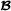along their common modes, as the tensor$\mathcal{C}\in {\mathbb{C}}^{{R}_{1}×\cdots ×{R}_{N}×{I}_{1}×\cdots ×{I}_{P}}$ such that

$\phantom{\rule{-13.0pt}{0ex}}\mathcal{C}\phantom{\rule{0.3em}{0ex}}=\phantom{\rule{0.3em}{0ex}}\mathcal{A}\phantom{\rule{1em}{0ex}}\underset{\mathbb{R}}{\odot }\phantom{\rule{0.3em}{0ex}}\mathcal{B}\phantom{\rule{0.3em}{0ex}}⇔\phantom{\rule{0.3em}{0ex}}{c}_{{r}_{1},\dots ,{r}_{N},{i}_{1},\dots ,{i}_{P}}\phantom{\rule{0.3em}{0ex}}=\phantom{\rule{0.3em}{0ex}}{a}_{{r}_{1},\dots ,{r}_{N},{i}_{1},\dots ,{i}_{{P}_{1}}}\phantom{\rule{0.3em}{0ex}}{b}_{{r}_{1},\dots ,{r}_{N},{i}_{{P}_{1}+1},\dots ,{i}_{P}}$

For instance, given two third‐order tensors$\mathcal{A}\in {\mathbb{C}}^{{R}_{1}×{R}_{2}×{I}_{1}}$ and$\mathcal{B}\in {\mathbb{C}}^{{R}_{1}×{R}_{2}×{I}_{2}}$, the Hadamard product$\mathcal{A}\phantom{\rule{0.3em}{0ex}}\underset{\left\{{r}_{1},{r}_{2}\right\}}{\odot }\phantom{\rule{0.3em}{0ex}}\mathcal{B}$ gives a fourth‐order tensor$\mathcal{C}\in {\mathbb{C}}^{{R}_{1}×{R}_{2}×{I}_{1}×{I}_{2}}$ such that

${c}_{{r}_{1},{r}_{2},{i}_{1},{i}_{2}}={a}_{{r}_{1},{r}_{2},{i}_{1}}{b}_{{r}_{1},{r}_{2},{i}_{2}}.$

Such a tensor Hadamard product can be calculated by means of the matrix Hadamard product of matrix unfoldings of extended tensors, as defined in (21) and (22) (see also (94) to (96) in Appendix 2). For the example above, we have

$\phantom{\rule{-5.0pt}{0ex}}{\mathbf{C}}_{{R}_{1}{R}_{2}×{I}_{1}{I}_{2}}={\mathbf{A}}_{{R}_{1}{R}_{2}×{I}_{1}}\left({\mathbf{I}}_{{I}_{1}}\otimes {\mathbf{1}}_{{I}_{2}}^{T}\right)\odot {\mathbf{B}}_{{R}_{1}{R}_{2}×{I}_{2}}\left({\mathbf{1}}_{{I}_{1}}^{T}\otimes {\mathbf{I}}_{{I}_{2}}\right)$
##### 1.2.1.0 Example

For${\mathbf{A}}_{R×{I}_{1}}=\left[\begin{array}{cc}{a}_{1}& {a}_{2}\\ {a}_{3}& {a}_{4}\end{array}\right]$,${\mathbf{B}}_{R×{I}_{2}}=\left[\begin{array}{cc}{b}_{1}& {b}_{2}\\ {b}_{3}& {b}_{4}\end{array}\right]$, and the tensor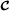such as${c}_{r,{i}_{1},{i}_{2}}={a}_{r,{i}_{1}}{b}_{r,{i}_{2}}$, a mode‐1 flat matrix unfolding ofis given by

$\begin{array}{ll}{\mathbf{C}}_{R×{I}_{1}{I}_{2}}& ={\mathbf{A}}_{R×{I}_{1}}\left({\mathbf{I}}_{2}\otimes {\mathbf{1}}_{2}^{T}\right)\odot {\mathbf{B}}_{R×{I}_{2}}\left({\mathbf{1}}_{2}^{T}\otimes {\mathbf{I}}_{2}\right)\phantom{\rule{2em}{0ex}}\\ =\left[\begin{array}{cccc}{a}_{1}& {a}_{1}& {a}_{2}& {a}_{2}\\ {a}_{3}& {a}_{3}& {a}_{4}& {a}_{4}\end{array}\right]\odot \left[\begin{array}{cccc}{b}_{1}& {b}_{2}& {b}_{1}& {b}_{2}\\ {b}_{3}& {b}_{4}& {b}_{3}& {b}_{4}\end{array}\right]\phantom{\rule{2em}{0ex}}\\ =\left[\begin{array}{cccc}{a}_{1}{b}_{1}& {a}_{1}{b}_{2}& {a}_{2}{b}_{1}& {a}_{2}{b}_{2}\\ {a}_{3}{b}_{3}& {a}_{3}{b}_{4}& {a}_{4}{b}_{3}& {a}_{4}{b}_{4}\end{array}\right]\phantom{\rule{2em}{0ex}}\end{array}$

#### 1.2.2 Mode combination

Different contraction operations can be defined depending on the way according to which the modes are combined. Let us partition the set {1,…,N} in N1 ordered subsets${\mathbb{S}}_{{n}_{1}}$, constituted of p(n1) elements with$\sum _{{n}_{1}=1}^{{N}_{1}}p\left({n}_{1}\right)=N$. Each subset${\mathbb{S}}_{{n}_{1}}$ is associated with a combined mode of dimension${J}_{{n}_{1}}=\underset{n\in {\mathbb{S}}_{{n}_{1}}}{\prod {I}_{n}}$. These mode combinations allow to rewrite the N th‐order tensor$\mathcal{X}\in {\mathbb{C}}^{{I}_{1}×\cdots ×{I}_{N}}$ under the form of an${N}_{1}^{\text{th}}$‐order tensor$\mathcal{Y}\in {\mathcal{C}}^{{J}_{1}×\cdots ×{J}_{{N}_{1}}}$ as follows

$\phantom{\rule{-10.0pt}{0ex}}\mathcal{Y}=\sum _{{j}_{1}=1}^{{J}_{1}}\phantom{\rule{0.3em}{0ex}}\cdots \phantom{\rule{0.3em}{0ex}}\sum _{{j}_{{N}_{1}}=1}^{{J}_{{N}_{1}}}\phantom{\rule{0.3em}{0ex}}{x}_{{j}_{1},\dots ,{j}_{{N}_{1}}}\stackrel{{N}_{1}}{\underset{{n}_{1}=1}{\circ }}{\mathbf{e}}_{{j}_{{n}_{1}}}^{\left({J}_{{n}_{1}}\right)}\phantom{\rule{0.3em}{0ex}}\phantom{\rule{0.3em}{0ex}}\text{with}\phantom{\rule{0.3em}{0ex}}\phantom{\rule{0.3em}{0ex}}{\mathbf{e}}_{{j}_{{n}_{1}}}^{\left({J}_{{n}_{1}}\right)}=\underset{n\in {\mathbb{S}}_{{n}_{1}}}{\otimes }\phantom{\rule{0.3em}{0ex}}{\mathbf{e}}_{{i}_{n}}^{\left({I}_{n}\right)}.$
(5)

Two particular mode combinations corresponding to the vectorization and matricization operations are now detailed.

#### 1.2.3 Vectorization

The vectorization of$\mathcal{X}\in {\mathbb{C}}^{{I}_{1}×\cdots ×{I}_{N}}$ is associated with a combination of the N modes into a unique mode of dimension$J=\prod _{n=1}^{N}{I}_{n}$, which amounts to replace the outer product in (4) by the Kronecker product

$\text{vec}\left(\mathcal{X}\right)=\sum _{{i}_{1}=1}^{{I}_{1}}\cdots \sum _{{i}_{N}=1}^{{I}_{N}}{x}_{{i}_{1},\dots ,{i}_{N}}\stackrel{N}{\underset{n=1}{\otimes }}{\mathbf{e}}_{{i}_{n}}^{\left({I}_{n}\right)}\in {\mathbb{C}}^{{I}_{1}\cdots {I}_{N}×1}$
(6)

the element${x}_{{i}_{1},\dots ,{i}_{N}}$ ofbeing the i th entry of$\text{vec}\left(\mathcal{X}\right)$ with i defined as in (3).

The vectorization can also be carried out after a permutation of indices π(i n ),n=1,…,N.

#### 1.2.4 Matricization or unfolding

There are different ways of matricizing the tensoraccording to the partitioning of the set {1,…,N} into two ordered subsets${\mathbb{S}}_{1}$ and${\mathbb{S}}_{2}$, constituted of p and N-p indices, respectively. A general formula for the matricization, for$p\in \left[1,N-1\right]$, is

$\phantom{\rule{-15.0pt}{0ex}}{\mathbf{X}}_{{\mathbb{S}}_{1};{\mathbb{S}}_{2}}=\phantom{\rule{0.3em}{0ex}}\sum _{{i}_{1}=1}^{{I}_{1}}\phantom{\rule{0.3em}{0ex}}\cdots \phantom{\rule{0.3em}{0ex}}\sum _{{i}_{N}=1}^{{I}_{N}}\phantom{\rule{0.3em}{0ex}}{x}_{{i}_{1},\dots ,{i}_{N}}\left(\underset{n\in {\mathbb{S}}_{1}}{\otimes }{\mathbf{e}}_{{i}_{n}}^{\left({I}_{n}\right)}\phantom{\rule{0.3em}{0ex}}\right){\left(\underset{n\in {\mathbb{S}}_{2}}{\otimes }{\mathbf{e}}_{{i}_{n}}^{\left({I}_{n}\right)}\phantom{\rule{0.3em}{0ex}}\right)}^{T}\phantom{\rule{0.3em}{0ex}}\phantom{\rule{0.3em}{0ex}}\in \phantom{\rule{0.3em}{0ex}}{\mathbb{C}}^{{J}_{1}×{J}_{2}}$
(7)

with${J}_{{n}_{1}}=\underset{n\in {\mathbb{S}}_{{n}_{1}}}{\prod {I}_{n}}$, for n1=1 and 2. From (7), we can deduce the following expression of the element${x}_{{i}_{1},\dots ,{i}_{N}}$ in terms of the matrix unfolding${\mathbf{X}}_{{\mathbb{S}}_{1};{\mathbb{S}}_{2}}$

${x}_{{i}_{1},\dots ,{i}_{N}}={\left(\underset{n\in {\mathbb{S}}_{1}}{\otimes }{\mathbf{e}}_{{i}_{n}}^{\left({I}_{n}\right)}\right)}^{T}{\mathbf{X}}_{{\mathbb{S}}_{1};{\mathbb{S}}_{2}}\left(\underset{n\in {\mathbb{S}}_{2}}{\otimes }{\mathbf{e}}_{{i}_{n}}^{\left({I}_{n}\right)}\right).$
(8)

#### 1.2.5 Particular case: mode‐ n matrix unfoldings X n

A flat mode‐n matrix unfolding of the tensorcorresponds to an unfolding under the form${\mathbf{X}}_{{\mathbb{S}}_{1};{\mathbb{S}}_{2}}$ with${\mathbb{S}}_{1}=\left\{n\right\}$ and${\mathbb{S}}_{2}=\left\{n+1,\dots ,N,1,\dots ,n-1\right\}$, which gives

$\begin{array}{l}{\mathbf{X}}_{{I}_{n}×{I}_{n+1}\cdots {I}_{N}{I}_{1}\cdots {I}_{n-1}}={\mathbf{X}}_{n}\\ =\phantom{\rule{0.3em}{0ex}}\sum _{{i}_{1}=1}^{{I}_{1}}\phantom{\rule{0.3em}{0ex}}\cdots \phantom{\rule{0.3em}{0ex}}\sum _{{i}_{N}=1}^{{I}_{N}}{x}_{{i}_{1},\dots ,{i}_{N}}{\mathbf{e}}_{{i}_{n}}^{\left({I}_{n}\right)}{\left(\underset{n\in {\mathbb{S}}_{2}}{\otimes }{\mathbf{e}}_{{i}_{n}}^{\left({I}_{n}\right)}\phantom{\rule{0.3em}{0ex}}\right)}^{T}\phantom{\rule{0.3em}{0ex}}\in \phantom{\rule{0.3em}{0ex}}{\mathbb{C}}^{{I}_{n}\phantom{\rule{0.3em}{0ex}}×\phantom{\rule{0.3em}{0ex}}{I}_{n+1}\cdots {I}_{N}{I}_{1}\cdots {I}_{n-1}}.\end{array}$
(9)

We can also define a tall mode‐n matrix unfolding of, by choosing${\mathbb{S}}_{1}=\left\{n+1,\dots ,N,1,\dots ,n-1\right\}$ and${\mathbb{S}}_{2}=\left\{n\right\}$. Then, we have${\mathbf{X}}_{{I}_{n+1}\cdots {I}_{N}{I}_{1}\cdots {I}_{n-1}×{I}_{n}}={\mathbf{X}}_{n}^{T}\in {\mathbb{C}}^{{I}_{n+1}\cdots {I}_{N}{I}_{1}\cdots {I}_{n-1}×{I}_{n}}$.

The column vectors of a flat mode‐n matrix unfolding X n are the mode‐n vectors of, and the rank of X n , i.e., the dimension of the mode‐n linear space spanned by the mode‐n vectors, is called mode‐n rank of, denoted by${\text{rank}}_{n}\left(\mathcal{X}\right)$.

In the case of a third‐order tensor$\mathcal{X}\in {\mathbb{C}}^{I×J×K}$, there are six different flat unfoldings, denoted XI×J K, XI×K J, XJ×K I, XJ×I K, XK×I J, and XK×J I. For instance, we have

$\phantom{\rule{-10.0pt}{0ex}}{\mathbf{X}}_{I×\mathit{\text{JK}}}={\mathbf{X}}_{\left\{1\right\};\left\{2,3\right\}}=\sum _{i=1}^{I}\sum _{j=1}^{J}\sum _{k=1}^{K}{x}_{i,j,k}\phantom{\rule{0.3em}{0ex}}{\mathbf{e}}_{i}^{\left(I\right)}{\left({\mathbf{e}}_{j}^{\left(J\right)}\otimes {\mathbf{e}}_{k}^{\left(K\right)}\right)}^{T}.$
(10)

Using the properties (84), (85), and (87) of the Kronecker product gives

$\begin{array}{ll}\phantom{\rule{-12.0pt}{0ex}}{\mathbf{X}}_{I×\mathit{\text{JK}}}& =\sum _{j=1}^{J}{\left({\mathbf{e}}_{j}^{\left(J\right)}\right)}^{T}\otimes \sum _{i=1}^{I}\sum _{k=1}^{K}{x}_{i,j,k}{\mathbf{e}}_{i}^{\left(I\right)}{\left({\mathbf{e}}_{k}^{\left(K\right)}\right)}^{T}\phantom{\rule{2em}{0ex}}\\ =\sum _{j=1}^{J}{\left({\mathbf{e}}_{j}^{\left(J\right)}\right)}^{T}\otimes {\left({\mathbf{X}}_{\mathrm{.j.}}\right)}^{T}=\left[\begin{array}{ccc}{\mathbf{X}}_{.1.}^{T}& \cdots & {\mathbf{X}}_{\mathrm{.J.}}^{T}\end{array}\right]\in {\mathbb{C}}^{I×\mathit{\text{JK}}}.\phantom{\rule{2em}{0ex}}\end{array}$

Similarly, there are six tall matrix unfoldings, denoted XJ K×I, XK J×I, XK I×J, XI K×J, XI J×K, XJ I×K, like for instance

$\phantom{\rule{-15.0pt}{0ex}}{\mathbf{X}}_{\mathit{\text{JK}}×I}=\phantom{\rule{0.3em}{0ex}}\sum _{i=1}^{I}\sum _{j=1}^{J}\sum _{k=1}^{K}\phantom{\rule{0.3em}{0ex}}{x}_{i,j,k}\phantom{\rule{0.3em}{0ex}}\left({\mathbf{e}}_{j}^{\left(J\right)}\phantom{\rule{0.3em}{0ex}}\otimes \phantom{\rule{0.3em}{0ex}}{\mathbf{e}}_{k}^{\left(K\right)}\right)\phantom{\rule{0.3em}{0ex}}{{\mathbf{e}}_{i}^{\left(I\right)}}^{T}\phantom{\rule{0.3em}{0ex}}=\phantom{\rule{0.3em}{0ex}}{\mathbf{X}}_{I×\mathit{\text{JK}}}^{T}\phantom{\rule{0.3em}{0ex}}\in \phantom{\rule{0.3em}{0ex}}{\mathbb{C}}^{\mathit{\text{JK}}×I}.$
(11)

Applying (8) to (10) gives

$\phantom{\rule{-10.0pt}{0ex}}{x}_{i,j,k}={\left({\mathbf{e}}_{i}^{\left(I\right)}\right)}^{T}{\mathbf{X}}_{I×\mathit{\text{JK}}}\left({\mathbf{e}}_{j}^{\left(J\right)}\otimes {\mathbf{e}}_{k}^{\left(K\right)}\right)={\left[{\mathbf{X}}_{I×\mathit{\text{JK}}}\right]}_{i,\left(j-1\right)K+k}.$

#### 1.2.6 Mode‐ n product of a tensor with a matrix or a vector

The mode‐n product of$\mathcal{X}\in {\mathbb{C}}^{{I}_{1}×\cdots ×{I}_{N}}$ with$\mathbf{A}\in {\mathbb{C}}^{{J}_{n}×{I}_{n}}$ along the n th mode, denoted by$\mathcal{X}{×}_{n}\mathbf{A}$, gives the tensor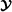of order N and dimensions I1××In-1×J n ×In+1××I N , such as

${y}_{{i}_{1},\dots ,{i}_{n-1},{j}_{n},{i}_{n+1},\dots ,{i}_{N}}=\sum _{{i}_{n}=1}^{{I}_{n}}{a}_{{j}_{n},{i}_{n}}{x}_{{i}_{1},\dots ,{i}_{n-1},{i}_{n},{i}_{n+1},\dots ,{i}_{N}}$
(12)

which can be expressed in terms of mode‐n matrix unfoldings ofand${\mathbf{Y}}_{n}={\mathbf{AX}}_{n}.$

This operation can be interpreted as the linear map from the mode‐n space ofto the mode‐n space of, associated with the matrix A.

The mode‐n product of$\mathcal{X}\in {\mathbb{C}}^{{I}_{1}×\cdots ×{I}_{N}}$ with the row vector${\mathbf{u}}^{T}\in {\mathbb{C}}^{1×{I}_{n}}$ along the n th mode, denoted by$\mathcal{X}{×}_{n}{\mathbf{u}}^{T}$, gives a tensorof order N-1 and dimensions I1××In-1×In+1××I N , such as

${y}_{{i}_{1},\dots ,{i}_{n-1},{i}_{n+1},\dots ,{i}_{N}}=\sum _{{i}_{n}=1}^{{I}_{n}}{u}_{{i}_{n}}{x}_{{i}_{1},\dots ,{i}_{n-1},{i}_{n},{i}_{n+1},\dots ,{i}_{N}}$

that can be written in vectorized form as${\text{vec}}^{T}\left(\mathcal{Y}\right)={\mathbf{u}}^{T}{\mathbf{X}}_{n}\in {\mathbb{C}}^{1×{I}_{n+1}\cdots {I}_{N}{I}_{1}\cdots {I}_{n-1}}$.

When multiplying a N th‐order tensor by row vectors along p different modes, we get a tensor of order N-p. For instance, for a third‐order tensor$\mathcal{X}\in {\mathbb{C}}^{I×J×K}$, we have

$\phantom{\rule{-15.0pt}{0ex}}{\mathbf{x}}_{\mathrm{ij.}}\phantom{\rule{0.3em}{0ex}}=\phantom{\rule{0.3em}{0ex}}\mathcal{X}{×}_{1}{{\mathbf{e}}_{i}^{\left(I\right)}}^{T}\phantom{\rule{0.3em}{0ex}}{×}_{2}\phantom{\rule{0.3em}{0ex}}{{\mathbf{e}}_{j}^{\left(J\right)}}^{T},\phantom{\rule{1em}{0ex}}\phantom{\rule{0.3em}{0ex}}{x}_{\mathit{\text{ijk}}}\phantom{\rule{0.3em}{0ex}}=\phantom{\rule{0.3em}{0ex}}\mathcal{X}{×}_{1}{{\mathbf{e}}_{i}^{\left(I\right)}}^{T}\phantom{\rule{0.3em}{0ex}}{×}_{2}\phantom{\rule{0.3em}{0ex}}{{\mathbf{e}}_{j}^{\left(J\right)}}^{T}\phantom{\rule{0.3em}{0ex}}{×}_{3}\phantom{\rule{0.3em}{0ex}}{{\mathbf{e}}_{k}^{\left(K\right)}}^{T}.$

Considering an ordered subset$\mathbb{S}\phantom{\rule{0.3em}{0ex}}=\phantom{\rule{0.3em}{0ex}}\left\{{m}_{1},\dots ,{m}_{P}\right\}$ of the set {1,…,N}, a series of mode‐ m p products of$\mathcal{X}\in {\mathbb{C}}^{{I}_{1}×\cdots ×{I}_{N}}$ with${\mathbf{A}}^{\left({m}_{p}\right)}\in {\mathbb{C}}^{{J}_{{m}_{p}}×{I}_{{m}_{p}}}$, p{1,…,P}, PN, will be concisely noted as

$\mathcal{X}{×}_{{m}_{1}}{\mathbf{A}}^{\left({m}_{1}\right)}\cdots {×}_{{m}_{P}}{\mathbf{A}}^{\left({m}_{P}\right)}=\mathcal{X}{×}_{m={m}_{1}}^{{m}_{P}}{\mathbf{A}}^{\left(m\right)}.$
##### 1.2.6.0 Properties
• For any permutation π(.) of P distinct indices m p {1,…,N} such as q p =π(m p ), p{1,…,P}, with PN, we have

$\mathcal{X}{×}_{q={q}_{1}}^{{q}_{P}}{\mathbf{A}}^{\left(q\right)}=\mathcal{X}{×}_{m={m}_{1}}^{{m}_{P}}{\mathbf{A}}^{\left(m\right)}$

which means that the order of the mode‐ m p products is irrelevant when the indices m p are all distinct.

• For two products of$\mathcal{X}\in {\mathbb{C}}^{{I}_{1}×\cdots ×{I}_{N}}$ along the same mode‐n, with$\mathbf{A}\in {\mathbb{C}}^{{J}_{n}×{I}_{n}}$ and$\mathbf{B}\in {\mathbb{C}}^{{K}_{n}×{J}_{n}}$, we have

$\begin{array}{l}\mathcal{Y}=\mathcal{X}{×}_{n}\mathbf{A}{×}_{n}\mathbf{B}\\ \phantom{\rule{0.5em}{0ex}}=\mathcal{X}{×}_{n}\left(\mathbf{B}\mathbf{A}\right)\in {\mathbb{C}}^{{I}_{1}×\cdots ×{I}_{n-1}×{K}_{n}×{I}_{n+1}×\cdots ×{I}_{N}}.\end{array}$
(13)

#### 1.2.7 Kronecker product‐based approach using index notation

In this subsection, we propose to reformulate our Kronecker product‐based approach for tensor matricization in terms of an index notation introduced in. Using this index notation, for$\mathbf{u}\in {\mathbb{C}}^{I×1}$,${\mathbf{v}}^{T}\in {\mathbb{C}}^{1×J}$, and$\mathbf{X}\in {\mathbb{C}}^{I×J}$, we can write

$\begin{array}{ll}\mathbf{u}& =\sum _{i=1}^{I}{u}_{i}{\mathbf{e}}_{i}^{\left(I\right)}={u}_{i}{\mathbf{e}}_{i}\phantom{\rule{2em}{0ex}}\\ {\mathbf{v}}^{T}& =\sum _{j=1}^{J}{v}_{j}{\left({\mathbf{e}}_{j}^{\left(J\right)}\right)}^{T}={v}_{j}{\mathbf{e}}^{j}\phantom{\rule{2em}{0ex}}\\ \mathbf{X}& =\sum _{i=1}^{I}\sum _{j=1}^{j}{x}_{\mathit{\text{ij}}}\left({\mathbf{e}}_{i}^{\left(I\right)}\otimes {\left({\mathbf{e}}_{j}^{\left(J\right)}\right)}^{T}\right)={x}_{\mathit{\text{ij}}}{\mathbf{e}}_{i}^{j}\phantom{\rule{2em}{0ex}}\\ {\mathbf{X}}^{T}& ={x}_{\mathit{\text{ij}}}{\mathbf{e}}_{j}^{i}\phantom{\rule{2em}{0ex}}\\ \text{vec}\left(\mathbf{X}\right)& ={x}_{\mathit{\text{ij}}}{\mathbf{e}}_{\mathit{\text{ji}}}\phantom{\rule{2em}{0ex}}\end{array}$

As with Einstein summation convention, the index notation allows to drop summation signs. If an index i[ 1,I] is repeated in an expression (or more generally in a term of an equation), it means that this expression (or this term) must be summed over that index from 1 to I. However, it is worth noting the two differences between the index notation used in this paper and Einstein summation convention: (i) each index can be repeated more than twice in any expression and (i i) the index notation can be used with ordered sets of indices. We have to notice that the index notation can be interpreted in terms of two separate combinations of indices, one associated with the column (superscript) indices and the other one with the row (subscript) indices, with the following rules:

• the ordering of the column indices is independent of that of the row indices;

• the ordering of the column and row indices cannot be changed.

Considering the set$\mathbb{S}=\left\{{n}_{1},\dots ,{n}_{N}\right\}$ obtained by permuting the elements of {1,…,N} and defining the ordered set of indices$\mathbb{I}=\left\{{i}_{{n}_{1}},\dots ,{i}_{{n}_{N}}\right\}$ associated with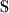, we denote by${\mathbf{e}}_{\mathbb{I}}$ and${\mathbf{e}}^{\mathbb{I}}$ the Kronecker products$\underset{n\in \mathbb{S}}{\otimes }{\mathbf{e}}_{{i}_{n}}$ and$\underset{n\in \mathbb{S}}{\otimes }{\mathbf{e}}_{{i}_{n}}^{T}$, respectively. So, we have

$\underset{n\in \mathbb{S}}{◇}{\mathbf{u}}^{\left(n\right)}=\left(\prod _{n\in \mathbb{S}}{u}_{{i}_{n}}^{\left(n\right)}\right){\mathbf{e}}_{\mathbb{I}}$
(14)

Partitioning two ordered sets of indices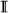and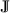into two subsets (${\mathbb{I}}_{1},{\mathbb{I}}_{2}$) and (${\mathbb{J}}_{1},{\mathbb{J}}_{2}$), respectively, the rules enounced previously imply the following identities:

$\begin{array}{ll}{\mathbf{e}}_{\mathbb{I}}^{\mathbb{J}}& ={\mathbf{e}}_{\mathbb{I}}\otimes {\mathbf{e}}^{\mathbb{J}}={\mathbf{e}}^{\mathbb{J}}\otimes {\mathbf{e}}_{\mathbb{I}}\phantom{\rule{0.3em}{0ex}}={\mathbf{e}}_{{\mathbb{I}}_{1}{\mathbb{I}}_{2}}^{{\mathbb{J}}_{1}{\mathbb{J}}_{2}}\phantom{\rule{2em}{0ex}}\\ ={\mathbf{e}}_{{\mathbb{I}}_{1}}\otimes {\mathbf{e}}_{{\mathbb{I}}_{2}}\otimes {\mathbf{e}}^{{\mathbb{J}}_{1}}\otimes {\mathbf{e}}^{{\mathbb{J}}_{2}}={\mathbf{e}}_{{\mathbb{I}}_{1}}\otimes {\mathbf{e}}^{{\mathbb{J}}_{1}}\otimes {\mathbf{e}}_{{\mathbb{I}}_{2}}\otimes {\mathbf{e}}^{{\mathbb{J}}_{2}}\phantom{\rule{2em}{0ex}}\\ ={\mathbf{e}}_{{\mathbb{I}}_{1}}\otimes {\mathbf{e}}^{{\mathbb{J}}_{1}}\otimes {\mathbf{e}}^{{\mathbb{J}}_{2}}\otimes {\mathbf{e}}_{{\mathbb{I}}_{2}}\phantom{\rule{2em}{0ex}}\\ ={\mathbf{e}}^{{\mathbb{J}}_{1}}\otimes {\mathbf{e}}^{{\mathbb{J}}_{2}}\otimes {\mathbf{e}}_{{\mathbb{I}}_{1}}\otimes {\mathbf{e}}_{{\mathbb{I}}_{2}}={\mathbf{e}}^{{\mathbb{J}}_{1}}\otimes {\mathbf{e}}_{{\mathbb{I}}_{1}}\otimes {\mathbf{e}}^{{\mathbb{J}}_{2}}\otimes {\mathbf{e}}_{{\mathbb{I}}_{2}}\phantom{\rule{2em}{0ex}}\\ ={\mathbf{e}}^{{\mathbb{J}}_{1}}\otimes {\mathbf{e}}_{{\mathbb{I}}_{1}}\otimes {\mathbf{e}}_{{\mathbb{I}}_{2}}\otimes {\mathbf{e}}^{{\mathbb{J}}_{2}}\phantom{\rule{2em}{0ex}}\end{array}$

These identities directly result from the property that the Kronecker product of a column vector with a row vector is independent of the order of the vectors (uvT=vTu), which implies that in a sequence of Kronecker products of column and row vectors, a column vector can be permuted with a row vector without altering the final result, if the proper ordering of the column vectors and of the row vectors is not changed in the sequence (${\mathbf{u}}_{1}\otimes {\mathbf{u}}_{2}\otimes {\mathbf{v}}^{T}={\mathbf{u}}_{1}\otimes {\mathbf{v}}^{T}\otimes {\mathbf{u}}_{2}={\mathbf{v}}^{T}\otimes {\mathbf{u}}_{1}\otimes {\mathbf{u}}_{2}\ne {\mathbf{v}}^{T}\otimes {\mathbf{u}}_{2}\otimes {\mathbf{u}}_{1}$ if u1u2).

Using the index notation, the horizontal, lateral, and frontal slices of a third‐order tensor$\mathcal{X}\in {\mathbb{C}}^{I×J×K}$ can be written as

${\mathbf{X}}_{\mathrm{i..}}={x}_{\mathit{\text{ijk}}}{\mathbf{e}}_{j}^{k}\phantom{\rule{0.3em}{0ex}}\phantom{\rule{0.3em}{0ex}};\phantom{\rule{0.3em}{0ex}}\phantom{\rule{0.3em}{0ex}}{\mathbf{X}}_{\mathrm{.j.}}={x}_{\mathit{\text{ijk}}}{\mathbf{e}}_{k}^{i}\phantom{\rule{0.3em}{0ex}}\phantom{\rule{0.3em}{0ex}};\phantom{\rule{0.3em}{0ex}}\phantom{\rule{0.3em}{0ex}}{\mathbf{X}}_{\mathrm{..k}}={x}_{\mathit{\text{ijk}}}{\mathbf{e}}_{i}^{j}.$

The Kronecker products of vectors ($\mathbf{u}\in {\mathbb{C}}^{I×1},\mathbf{v}\in {\mathbb{C}}^{J×1}$) and matrices ($\mathbf{A}\in {\mathbb{C}}^{I×J},\mathbf{B}\in {\mathbb{C}}^{K×L}$) can be concisely written as

$\begin{array}{ll}\mathbf{u}\otimes \mathbf{v}& =\left({u}_{i}{\mathbf{e}}_{i}\right)\otimes \left({v}_{j}{\mathbf{e}}_{j}\right)={u}_{i}{v}_{j}{\mathbf{e}}_{\mathit{\text{ij}}}\phantom{\rule{2em}{0ex}}\\ {\mathbf{u}}^{T}\otimes {\mathbf{v}}^{T}& ={u}_{i}{v}_{j}{\mathbf{e}}^{\mathit{\text{ij}}}\phantom{\rule{2em}{0ex}}\\ \mathbf{u}\otimes {\mathbf{v}}^{T}& ={u}_{i}{v}_{j}{\mathbf{e}}_{i}^{j}\phantom{\rule{2em}{0ex}}\\ \mathbf{A}\otimes \mathbf{B}& =\left({a}_{\mathit{\text{ij}}}{\mathbf{e}}_{i}^{j}\right)\otimes \left({b}_{\mathit{\text{kl}}}{\mathbf{e}}_{k}^{l}\right)={a}_{\mathit{\text{ij}}}{b}_{\mathit{\text{kl}}}{\mathbf{e}}_{\mathit{\text{ik}}}^{\mathit{\text{jl}}}\phantom{\rule{2em}{0ex}}\\ {\mathbf{A}}^{T}\otimes {\mathbf{B}}^{T}& ={a}_{\mathit{\text{ij}}}{b}_{\mathit{\text{kl}}}{\mathbf{e}}_{\mathit{\text{jl}}}^{\mathit{\text{ik}}}\phantom{\rule{2em}{0ex}}\end{array}$

For$\mathbf{U}=\left[{\mathbf{u}}^{\left(1\right)}\cdots {\mathbf{u}}^{\left(N\right)}\right]\in {\mathbb{C}}^{I×N}$ and$\mathbf{V}=\left[{\mathbf{v}}_{1}\cdots {\mathbf{v}}_{N}\right]\in {\mathbb{C}}^{J×N}$, we have

$\mathbf{U}{\mathbf{V}}^{T}=\sum _{n=1}^{N}{\mathbf{u}}^{\left(n\right)}{\left({\mathbf{v}}^{\left(n\right)}\right)}^{T}={u}_{i}^{\left(n\right)}{v}_{j}^{\left(n\right)}{\mathbf{e}}_{i}^{j}$
(15)

where the summation over n is to be done after the matricization u(n)(v(n))T.

Using the index notation, the Khatri‐Rao product can be written as follows:

$\begin{array}{cc}\mathbf{A}◇\mathbf{B}& ={a}_{\mathit{\text{ik}}}{b}_{\mathit{\text{jk}}}{\mathbf{e}}_{\mathit{\text{ij}}}^{k}\\ {\left(\mathbf{A}◇\mathbf{B}\right)}^{T}& ={a}_{\mathit{\text{ik}}}{b}_{\mathit{\text{jk}}}{\mathbf{e}}_{k}^{\mathit{\text{ij}}}\end{array}$
(16)

The Kronecker and Khatri‐Rao products defined in (1) and (2), with${a}_{{i}_{n},{r}_{n}}^{\left(n\right)}$ as entry of A(n), can then be defined as

$\begin{array}{ll}\underset{n\in \mathbb{S}}{\otimes }{\mathbf{A}}^{\left(n\right)}& =\left(\prod _{n\in \mathbb{S}}{a}_{{i}_{n},{r}_{n}}^{\left(n\right)}\right){\mathbf{e}}_{{i}_{{n}_{1}},\dots ,{i}_{{n}_{N}}}^{{r}_{{n}_{1}},\dots ,{r}_{{n}_{N}}}=\left(\prod _{n\in \mathbb{S}}{a}_{{i}_{n},{r}_{n}}^{\left(n\right)}\right){\mathbf{e}}_{\mathbb{I}}^{\mathbb{R}}\phantom{\rule{2em}{0ex}}\end{array}$
(17)
$\begin{array}{ll}\underset{n\in \mathbb{S}}{◇}{\mathbf{A}}^{\left(n\right)}& =\left(\prod _{n\in \mathbb{S}}{a}_{{i}_{n},r}^{\left(n\right)}\right){\mathbf{e}}_{{i}_{{n}_{1}},\dots ,{i}_{{n}_{N}}}^{r}=\left(\prod _{n\in \mathbb{S}}{a}_{{i}_{n},r}^{\left(n\right)}\right){\mathbf{e}}_{\mathbb{I}}^{r}\phantom{\rule{2em}{0ex}}\end{array}$
(18)

where$\mathbb{R}=\left\{{r}_{{n}_{1}},\dots ,{r}_{{n}_{N}}\right\}$.

Applying these results, the unfoldings (7), (10), and (11) and the formula (8) can be rewritten respectively as

$\begin{array}{ll}{\mathbf{X}}_{{\mathbb{S}}_{1};{\mathbb{S}}_{2}}& ={x}_{{i}_{1},\dots ,{i}_{N}}{\mathbf{e}}_{{\mathbb{I}}_{1}}^{{\mathbb{I}}_{2}}\phantom{\rule{2em}{0ex}}\end{array}$
(19)
$\begin{array}{ll}{\mathbf{X}}_{I×\mathit{\text{JK}}}& ={x}_{i,j,k}{\mathbf{e}}_{i}^{\mathit{\text{jk}}}\phantom{\rule{2em}{0ex}}\\ {\mathbf{X}}_{\mathit{\text{JK}}×I}& ={x}_{i,j,k}{\mathbf{e}}_{\mathit{\text{jk}}}^{i}\phantom{\rule{2em}{0ex}}\\ {x}_{{i}_{1},\dots ,{i}_{N}}& ={\mathbf{e}}^{{\mathbb{I}}_{1}}{\mathbf{X}}_{{\mathbb{S}}_{1};{\mathbb{S}}_{2}}{\mathbf{e}}_{{\mathbb{I}}_{2}}\phantom{\rule{2em}{0ex}}\end{array}$
(20)

where${\mathbb{I}}_{1}$ and${\mathbb{I}}_{2}$ represent the sets of indices i n associated with the sets${\mathbb{S}}_{1}$ and${\mathbb{S}}_{2}$ of index n, respectively.

We can also use the index notation for deriving matrix unfoldings of tensor extensions of a matrix$\mathbf{B}\in {\mathbb{C}}^{I×J}$. For instance, if we define the tensor$\mathcal{A}\in {\mathbb{C}}^{I×J×K}$ such as ai,j,k=bi,j for k=1,…,K, mode‐1 flat unfoldings ofare given by

$\begin{array}{ll}{\mathbf{A}}_{I×\mathit{\text{JK}}}& =\sum _{i,j,k}{a}_{i,j,k}{\mathbf{e}}_{i}\otimes {\mathbf{e}}^{j}\otimes {\mathbf{e}}^{k}={a}_{i,j,k}{\mathbf{e}}_{i}^{\mathit{\text{jk}}}\phantom{\rule{2em}{0ex}}\\ =\sum _{i,j}{b}_{i,j}{\mathbf{e}}_{i}^{j}\otimes \sum _{k=1}^{K}{\mathbf{e}}^{k}=\mathbf{B}\otimes {\mathbf{1}}_{K}^{T}=\mathbf{B}\left({\mathbf{I}}_{J}\otimes {\mathbf{1}}_{K}^{T}\right)\phantom{\rule{2em}{0ex}}\end{array}$
(21)
$\begin{array}{ll}{\mathbf{A}}_{I×\mathit{\text{KJ}}}& ={a}_{i,j,k}{\mathbf{e}}_{i}^{\mathit{\text{kj}}}=\sum _{k=1}^{K}{\mathbf{e}}^{k}\otimes {b}_{i,j}{\mathbf{e}}_{i}^{j}\phantom{\rule{2em}{0ex}}\\ ={\mathbf{1}}_{K}^{T}\otimes \mathbf{B}=\mathbf{B}\left({\mathbf{1}}_{K}^{T}\otimes {\mathbf{I}}_{J}\right)\phantom{\rule{2em}{0ex}}\end{array}$
(22)

These two formulae will be used later for establishing the link between PARATUCK‐(2,4) models and constrained PARAFAC‐4 models.

#### 1.2.8 Basic tensor models

We now present the two most common tensor models, i.e., the Tucker and PARAFAC models. In, these models are introduced in a constructive way, in the context of a three‐way data analysis. The Tucker models are presented as extensions of the matrix singular value decomposition (SVD) to three‐way arrays, which gave rise to the generalization as HOSVD[13, 49], whereas the PARAFAC model is introduced by emphasizing Cattell’s principle of parallel proportional profiles that underlies this model, so explaining the acronym PARAFAC. In the following, we adopt a more general presentation for multi‐way arrays, i.e., tensors of any order N.

##### 1.2.8.0 Tucker models

For a N th‐order tensor$\mathcal{X}\in {\mathbb{C}}^{{I}_{1}×\cdots ×{I}_{N}}$, a Tucker model is defined in an element‐wise form as

${x}_{{i}_{1},\dots ,{i}_{N}}=\sum _{{r}_{1}=1}^{{R}_{1}}\cdots \sum _{{r}_{N}=1}^{{R}_{N}}{g}_{{r}_{1},\dots ,{r}_{N}}\prod _{n=1}^{N}{a}_{{i}_{n},{r}_{n}}^{\left(n\right)}$
(23)

with i n =1,…,I n for n=1,…,N, where${g}_{{r}_{1},\dots ,{r}_{N}}$ is an element of the core tensor$\mathcal{G}\in {\mathbb{C}}^{{R}_{1}×\cdots ×{R}_{N}}$ and${a}_{{i}_{n},{r}_{n}}^{\left(n\right)}$ is an element of the matrix factor${\mathbf{A}}^{\left(n\right)}\in {\mathbb{C}}^{{I}_{n}×{R}_{n}}$.

Using the index notation and defining the set of indices$\mathbb{R}=\left\{{r}_{1},\dots ,{r}_{N}\right\}$, the Tucker model can also be written simply as

${x}_{{i}_{1},\dots ,{i}_{N}}={g}_{{r}_{1},\dots ,{r}_{N}}\prod _{\mathbb{R}}{a}_{{i}_{n},{r}_{n}}^{\left(n\right)}$
(24)

Taking the definition (4) into account and noting that$\sum _{{i}_{n}=1}^{{I}_{n}}{a}_{{i}_{n},{r}_{n}}^{\left(n\right)}{\mathbf{e}}_{{i}_{n}}^{\left({I}_{n}\right)}={\mathbf{A}}_{.{r}_{n}}^{\left(n\right)}$, this model can be written as a weighted sum of$\prod _{n=1}^{N}{R}_{n}$ outer products, i.e., rank‐one tensors

$\begin{array}{ll}\mathcal{X}& =\sum _{{r}_{1}=1}^{{R}_{1}}\cdots \sum _{{r}_{N}=1}^{{R}_{N}}{g}_{{r}_{1},\dots ,{r}_{N}}\stackrel{N}{\underset{n=1}{\circ }}{\mathbf{A}}_{.{r}_{n}}^{\left(n\right)}\phantom{\rule{2em}{0ex}}\\ ={g}_{{r}_{1},\dots ,{r}_{N}}\underset{\mathbb{R}}{\circ }{\mathbf{A}}_{.{r}_{n}}^{\left(n\right)}\phantom{\rule{0.3em}{0ex}}\phantom{\rule{0.3em}{0ex}}\left(\text{with the index notation}\right)\phantom{\rule{2em}{0ex}}\end{array}$
(25)

Using the definition (12) allows to write (23) in terms of mode‐n products as

$\begin{array}{l}\mathcal{X}=\mathcal{G}{×}_{1}{\mathbf{A}}^{\left(1\right)}{×}_{2}{\mathbf{A}}^{\left(2\right)}{×}_{3}\cdots {×}_{N}{\mathbf{A}}^{\left(N\right)}\\ \phantom{\rule{0.6em}{0ex}}=\mathcal{G}{×}_{n=1}^{N}{\mathbf{A}}^{\left(n\right)}.\end{array}$
(26)

This expression evidences that the Tucker model can be viewed as the transformation of the core tensor resulting from its multiplication by the factor matrix A(n) along its mode‐n, which corresponds to a linear map applied to the mode‐n space of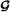, for n=1,…,N, i.e., a multilinear map applied to. From a transformation point of view,andcan be interpreted as the input tensor and the transformed tensor, or output tensor, respectively.

Matrix representations of the Tucker model. A matrix representation of a Tucker model is directly linked with a matricization of tensor like (7), corresponding to the combination of two sets of modes${\mathbb{S}}_{1}$ and${\mathbb{S}}_{2}$. These combinations must be applied both to the tensorand its core tensor.

The matrix representation (7) of the Tucker model (23) is given by

${\mathbf{X}}_{{\mathbb{S}}_{1};{\mathbb{S}}_{2}}=\left(\underset{n\in {\mathbb{S}}_{1}}{\otimes }{\mathbf{A}}^{\left(n\right)}\right){\mathbf{G}}_{{\mathbb{S}}_{1};{\mathbb{S}}_{2}}{\left(\underset{n\in {\mathbb{S}}_{2}}{\otimes }{\mathbf{A}}^{\left(n\right)}\right)}^{T}$
(27)

with${\mathbf{G}}_{{\mathbb{S}}_{1};{\mathbb{S}}_{2}}\in {\mathbb{C}}^{{J}_{1}×{J}_{2}}$, and${J}_{{n}_{1}}=\underset{n\in {\mathbb{S}}_{{n}_{1}}}{\prod {R}_{n}}$, for n1=1 and 2.

###### Proof

See Appendix 3.

For the flat mode‐n unfolding, defined in (9), the formula (27) gives

$\phantom{\rule{-15.0pt}{0ex}}{\mathbf{X}}_{n}={\mathbf{A}}^{\left(n\right)}{\mathbf{G}}_{n}{\left({\mathbf{A}}^{\left(n+1\right)}\phantom{\rule{0.3em}{0ex}}\otimes \phantom{\rule{0.3em}{0ex}}\cdots \phantom{\rule{0.3em}{0ex}}\otimes \phantom{\rule{0.3em}{0ex}}{\mathbf{A}}^{\left(N\right)}\phantom{\rule{0.3em}{0ex}}\otimes {\mathbf{A}}^{\left(1\right)}\phantom{\rule{0.3em}{0ex}}\otimes \phantom{\rule{0.3em}{0ex}}\cdots \phantom{\rule{0.3em}{0ex}}\otimes \phantom{\rule{0.3em}{0ex}}{\mathbf{A}}^{\left(n-1\right)}\right)}^{T}.$
(28)

Applying the vec formula (92) to the right‐hand side of (28), we obtain the vectorized form ofassociated with its mode‐n unfolding X n

$\begin{array}{l}\text{vec}\left(\mathcal{X}\right)=\text{vec}\left({\mathbf{X}}_{n}\right)\\ \phantom{\rule{2em}{0ex}}=\left({\mathbf{A}}^{\left(n+1\right)}\otimes \cdots \otimes {\mathbf{A}}^{\left(N\right)}\otimes {\mathbf{A}}^{\left(1\right)}\otimes \cdots \otimes {\mathbf{A}}^{\left(n\right)}\right)\text{vec}\left({\mathbf{G}}_{n}\right).\end{array}$
##### 1.2.8.0 Tucker‐(N 1 ,N) models

A Tucker‐ (N1,N) model for a N th‐order tensor$\mathcal{X}\in {\mathbb{C}}^{{I}_{1}×\cdots ×{I}_{N}}$, with NN1, corresponds to the case where N-N1 factor matrices are equal to identity matrices. For instance, assuming that${\mathbf{A}}^{\left(n\right)}={\mathbf{I}}_{{I}_{n}}$, which implies R n =I n , for n=N1+1,…,N, (23) and (26) become

$\begin{array}{ll}\phantom{\rule{-12.0pt}{0ex}}{x}_{{i}_{1},\dots ,{i}_{N}}& =\sum _{{r}_{1}=1}^{{R}_{1}}\cdots \sum _{{r}_{{N}_{1}}=1}^{{R}_{{N}_{1}}}{g}_{{r}_{1},\dots ,{r}_{{N}_{1}},{i}_{{N}_{1}+1},\dots ,{i}_{N}}\prod _{n=1}^{{N}_{1}}{a}_{{i}_{n},{r}_{n}}^{\left(n\right)}\phantom{\rule{2em}{0ex}}\\ \phantom{\rule{-12.0pt}{0ex}}\mathcal{X}& =\mathcal{G}{×}_{1}{\mathbf{A}}^{\left(1\right)}{×}_{2}\cdots {×}_{{N}_{1}}{\mathbf{A}}^{\left({N}_{1}\right)}{×}_{{N}_{1}+1}{\mathbf{I}}_{{I}_{{N}_{1}+1}}\cdots {×}_{N}{\mathbf{I}}_{{I}_{N}}\phantom{\rule{2em}{0ex}}\end{array}$
(29)
$\begin{array}{l}=\mathcal{G}\phantom{\rule{0.3em}{0ex}}{×}_{n=1}^{{N}_{1}}{\mathbf{A}}^{\left(n\right)}.\phantom{\rule{2em}{0ex}}\end{array}$
(30)

One such model that is currently used in applications is the Tucker‐(2,3) model, usually denoted Tucker2, for third‐order tensors$\mathcal{X}\in {\mathbb{C}}^{I×J×K}$. Assuming${\mathbf{A}}^{\left(1\right)}=\mathbf{A}\in {\mathbb{C}}^{I×P},{\mathbf{A}}^{\left(2\right)}=\mathbf{B}\in {\mathbb{C}}^{J×Q}$, and${\mathbf{A}}^{\left(3\right)}={\mathbf{I}}_{K}$, such a model is defined by the following equations:

$\begin{array}{ll}{x}_{\mathit{\text{ijk}}}& =\sum _{p=1}^{P}\sum _{q=1}^{Q}{g}_{\mathit{\text{pqk}}}{a}_{\mathit{\text{ip}}}{b}_{\mathit{\text{jq}}}\phantom{\rule{2em}{0ex}}\end{array}$
(31)
$\begin{array}{ll}\mathcal{X}& =\mathcal{G}{×}_{1}\mathbf{A}{×}_{2}\mathbf{B}\phantom{\rule{2em}{0ex}}\end{array}$
(32)

with the core tensor$\mathcal{G}\in {\mathbb{C}}^{P×Q×K}$.

##### 1.2.8.0 PARAFAC models

A PARAFAC model for a N th‐order tensor corresponds to the particular case of a Tucker model with an identity core tensor of order N and dimensions R××R

$\mathcal{G}={\mathcal{I}}_{N,R}=\mathcal{I}\phantom{\rule{1em}{0ex}}⇔\phantom{\rule{1em}{0ex}}{g}_{{r}_{1},\dots ,{r}_{N}}={\delta }_{{r}_{1},\dots ,{r}_{N}}$

(23) to (26) then become, respectively

$\begin{array}{ll}{x}_{{i}_{1},\dots ,{i}_{N}}& =\sum _{r=1}^{R}\prod _{n=1}^{N}{a}_{{i}_{n},r}^{\left(n\right)}\phantom{\rule{2em}{0ex}}\end{array}$
(33)
$\begin{array}{l}=\prod _{n=1}^{N}{a}_{{i}_{n},r}^{\left(n\right)}\phantom{\rule{0.3em}{0ex}}\phantom{\rule{0.3em}{0ex}}\phantom{\rule{0.3em}{0ex}}\phantom{\rule{0.3em}{0ex}}\phantom{\rule{0.3em}{0ex}}\phantom{\rule{0.3em}{0ex}}\left(\text{with the index notation}\right)\phantom{\rule{2em}{0ex}}\end{array}$
(34)
$\begin{array}{ll}\mathcal{X}& =\sum _{r=1}^{R}\left(\stackrel{N}{\underset{n=1}{\circ }}{\mathbf{A}}_{\mathrm{.r}}^{\left(n\right)}\right)\phantom{\rule{2em}{0ex}}\\ \mathcal{X}& ={\mathcal{I}}_{N,R}{×}_{n=1}^{N}{\mathbf{A}}^{\left(n\right)}\phantom{\rule{2em}{0ex}}\end{array}$
(35)

with the factor matrices${\mathbf{A}}^{\left(n\right)}\in {\mathbb{C}}^{{I}_{n}×R},n=1,\dots ,N$.

##### 1.2.8.0 Remarks
• The expression (33) as a sum of polyads is called a polyadic form ofby Hitchcock.

• The PARAFAC model (33, 34 and 35) amounts to decomposing the tensorinto a sum of R components, each component being a rank‐one tensor. When R is minimal in (33), it is called the rank of. This rank is related to the mode‐n ranks by the following inequalities${\text{rank}}_{n}\left(\mathcal{X}\right)\le R,\forall n=1,\dots ,N$. Furthermore, contrary to the matrices for which the rank is always at most equal to the smallest of the dimensions, for higher‐order tensors, the rank can exceed any mode‐n dimension I n .

• There exists different definitions of rank for tensors, like typical and generic ranks, or also symmetric rank for a symmetric tensor (see[53, 54] for more details).

• In telecommunication applications, the structure parameters (rank, mode dimensions, and core tensor dimensions) of a PARAFAC or Tucker model are design parameters that are chosen in function of the performance desired for the communication system. However, in most of the applications, as for instance in multi‐way data analysis, the structure parameters are generally unknown and must be determined a priori. Several techniques have been proposed for determining these parameters (see‐ and references therein).

• The PARAFAC model is also sometimes defined by the following equation

${x}_{{i}_{1},\dots ,{i}_{N}}=\sum _{r=1}^{R}{g}_{r}\prod _{n=1}^{N}{a}_{{i}_{n},r}^{\left(n\right)}\phantom{\rule{1em}{0ex}}\text{with}\phantom{\rule{1em}{0ex}}{g}_{r}>0.$
(36)
• In this case, the identity tensor${\mathcal{I}}_{N,R}$ in (35) is replaced by the diagonal tensor$\mathcal{G}\in {\mathbb{C}}^{R×\cdots ×R}$ whose diagonal elements are equal to scaling factors g r , i.e.

${g}_{{r}_{1},\dots ,{r}_{N}}=\left\{\begin{array}{l}{g}_{r}\phantom{\rule{1em}{0ex}}\text{if}\phantom{\rule{1em}{0ex}}{r}_{1}=\cdots ={r}_{N}=r\\ 0\phantom{\rule{1em}{0ex}}\text{otherwise}\end{array}\right\$

and all the column vectors${\mathbf{A}}_{\mathrm{.r}}^{\left(n\right)}$ are normalized, i.e., with a unit norm, for 1≤nN.

• It is important to notice that the PARAFAC model (33) is multilinear (more precisely N‐linear) in its parameters in the sense that it is linear with respect to each matrix factor. This multilinearity property is exploited for parameter estimation using the standard alternating least squares (ALS) algorithm[4, 5] that consists in alternately estimating each matrix factor by minimizing a least squares error criterion conditionally to the knowledge of the other matrix factors that are fixed with their previously estimated values.

Matrix representations of the PARAFAC model. The matrix representation (7) of the PARAFAC model (33)‐(35) is given by

${\mathbf{X}}_{{\mathbb{S}}_{1};{\mathbb{S}}_{2}}=\left(\underset{n\in {\mathbb{S}}_{1}}{◇}{\mathbf{A}}^{\left(n\right)}\right){\left(\underset{n\in {\mathbb{S}}_{2}}{◇}{\mathbf{A}}^{\left(n\right)}\right)}^{T}.$
(37)

See Appendix 4.

##### 1.2.8.0 Remarks
• From (37), we can deduce that a mode combination results in a Khatri‐Rao product of the corresponding factor matrices. Consequently, the tensor contraction (5) associated with the PARAFAC‐N model (35) gives a PARAFAC‐ N1 model whose factor matrices are equal to$\underset{n\in {\mathbb{S}}_{{n}_{1}}}{◇}{\mathbf{A}}^{\left(n\right)}\in {\mathbb{C}}^{{J}_{{n}_{1}}×R}$, n1=1,…,N1, with${J}_{{n}_{1}}=\underset{n\in {\mathbb{S}}_{{n}_{1}}}{\prod {I}_{n}}$.

• For the PARAFAC model, the flat mode‐n unfolding, defined in (9), is given by

$\phantom{\rule{-5.0pt}{0ex}}{\mathbf{X}}_{n}={\mathbf{A}}^{\left(n\right)}{\left({\mathbf{A}}^{\left(n+1\right)}◇\cdots ◇{\mathbf{A}}^{\left(N\right)}◇{\mathbf{A}}^{\left(1\right)}◇\cdots ◇{\mathbf{A}}^{\left(n-1\right)}\right)}^{T},$
(38)
• and the associated vectorized form is obtained in applying the vec formula (93) to the right‐hand side of the above equation, with I R =diag(1 R )

$\phantom{\rule{-15.0pt}{0ex}}\text{vec}\left(\mathcal{X}\right)=\text{vec}\left({\mathbf{X}}_{n}\right)=\left({\mathbf{A}}^{\left(n+1\right)}\phantom{\rule{0.3em}{0ex}}◇\phantom{\rule{0.3em}{0ex}}\cdots \phantom{\rule{0.3em}{0ex}}◇\phantom{\rule{0.3em}{0ex}}{\mathbf{A}}^{\left(N\right)}\phantom{\rule{0.3em}{0ex}}◇\phantom{\rule{0.3em}{0ex}}{\mathbf{A}}^{\left(1\right)}\phantom{\rule{0.3em}{0ex}}◇\phantom{\rule{0.3em}{0ex}}\cdots \phantom{\rule{0.3em}{0ex}}◇\phantom{\rule{0.3em}{0ex}}{\mathbf{A}}^{\left(n\right)}\right){\mathbf{1}}_{R}$
(39)
• In the case of the normalized PARAFAC model (36), (37) and (39) become, respectively,

$\begin{array}{ll}\phantom{\rule{-10.0pt}{0ex}}{\mathbf{X}}_{{\mathbb{S}}_{1};{\mathbb{S}}_{2}}& =\left(\underset{n\in {\mathbb{S}}_{1}}{◇}{\mathbf{A}}^{\left(n\right)}\right)\text{diag}\left(\mathbf{g}\right){\left(\underset{n\in {\mathbb{S}}_{2}}{◇}{\mathbf{A}}^{\left(n\right)}\right)}^{T}\phantom{\rule{2em}{0ex}}\\ \phantom{\rule{-10.0pt}{0ex}}\text{vec}\left(\mathcal{X}\right)& =\text{vec}\left({\mathbf{X}}_{n}\right)\phantom{\rule{2em}{0ex}}\\ =\left({\mathbf{A}}^{\left(n+1\right)}◇\cdots ◇{\mathbf{A}}^{\left(N\right)}◇{\mathbf{A}}^{\left(1\right)}◇\cdots ◇{\mathbf{A}}^{\left(n\right)}\right)\phantom{\rule{0.3em}{0ex}}\mathbf{g}\phantom{\rule{2em}{0ex}}\end{array}$
• where$\mathbf{g}={\left[{g}_{1}\cdots {g}_{R}\right]}^{T}\in {\mathbb{C}}^{R×1}$.

• For the PARAFAC model of a third‐order tensor$\mathcal{X}\in {\mathbb{C}}^{I×J×K}$ with factor matrices (A,B,C), the formula (37) gives for${\mathbb{S}}_{1}=\left\{i,j\right\}$ and${\mathbb{S}}_{2}=\left\{k\right\}$

${\mathbf{X}}_{\mathit{\text{IJ}}×K}=\left[\begin{array}{c}{\mathbf{X}}_{1\mathrm{..}}\\ ⋮\\ {\mathbf{X}}_{\mathrm{I..}}\end{array}\right]=\left(\mathbf{A}◇\mathbf{B}\right){\mathbf{C}}^{T}\in {\mathbb{C}}^{\mathit{\text{IJ}}×K}.$
• Noting that$\mathbf{A}◇\mathbf{B}=\left[\begin{array}{c}\mathbf{B}{D}_{1}\left(\mathbf{A}\right)\\ ⋮\\ \mathbf{B}{D}_{I}\left(\mathbf{A}\right)\end{array}\right]$, we deduce the following expression for mode‐1 matrix slices

${\mathbf{X}}_{\mathrm{i..}}=\mathbf{B}{D}_{i}\left(\mathbf{A}\right){\mathbf{C}}^{T}.$
• Similarly, we have

$\begin{array}{ll}{\mathbf{X}}_{\mathit{\text{JK}}×I}& =\left(\mathbf{B}◇\mathbf{C}\right){\mathbf{A}}^{T},\phantom{\rule{1em}{0ex}}{\mathbf{X}}_{\mathit{\text{KI}}×J}=\left(\mathbf{C}◇\mathbf{A}\right){\mathbf{B}}^{T},\phantom{\rule{2em}{0ex}}\\ {\mathbf{X}}_{\mathrm{.j.}}& =\mathbf{C}{D}_{j}\left(\mathbf{B}\right){\mathbf{A}}^{T},\phantom{\rule{1em}{0ex}}{\mathbf{X}}_{\mathrm{..k}}=\mathbf{A}{D}_{k}\left(\mathbf{C}\right){\mathbf{B}}^{T}.\phantom{\rule{2em}{0ex}}\end{array}$
• For the PARAFAC model of a fourth‐order tensor$\mathcal{X}\in {\mathbb{C}}^{I×J×K×L}$ with factor matrices (A,B,C,D), we obtain

$\begin{array}{ll}{\mathbf{X}}_{\mathit{\text{IJK}}×L}& =\left(\mathbf{A}◇\mathbf{B}◇\mathbf{C}\right){\mathbf{D}}^{T}\phantom{\rule{2em}{0ex}}\\ =\left[\begin{array}{c}\left(\mathbf{B}◇\mathbf{C}\right){D}_{1}\left(\mathbf{A}\right)\\ ⋮\\ \left(\mathbf{B}◇\mathbf{C}\right){D}_{I}\left(\mathbf{A}\right)\end{array}\right]{\mathbf{D}}^{T}\phantom{\rule{2em}{0ex}}\\ =\left[\begin{array}{c}\mathbf{C}{D}_{1}\left(\mathbf{B}\right){D}_{1}\left(\mathbf{A}\right)\\ ⋮\\ \mathbf{C}{D}_{J}\left(\mathbf{B}\right){D}_{I}\left(\mathbf{A}\right)\end{array}\right]{\mathbf{D}}^{T}\in {\mathbb{C}}^{\mathit{\text{IJK}}×L}\phantom{\rule{2em}{0ex}}\\ {\mathbf{X}}_{\mathrm{ij..}}& =\mathbf{C}{D}_{j}\left(\mathbf{B}\right){D}_{i}\left(\mathbf{A}\right){\mathbf{D}}^{T}\in {\mathbb{C}}^{K×L}\phantom{\rule{2em}{0ex}}\end{array}$
(40)

Other matrix slices can be deduced from (40) by simple permutations of the matrix factors.

In the next section, we introduce two constrained PARAFAC models, the so‐called PARALIND and CONFAC models, and then PARATUCK models.

### 1.3 Constrained PARAFAC models

The introduction of constraints in tensor models can result from the system itself that is under study or from a system design. In the first case, the constraints are often interpreted as interactions or linear dependencies between the PARAFAC factors. Examples of such dependencies are encountered in psychometric and chemometric applications that gave origin, respectively, to the PARATUCK‐2 model and the parallel profiles with linear dependencies (PARALIND) model[60, 61], introduced in under the name canonical decomposition with linear constraints (CANDELINC), for the multiway case. A first application of the PARATUCK‐2 model in signal processing was made in for blind joint identification and equalization of Wiener‐Hammerstein communication channels. The PARALIND model was applied for identifiability and propagation parameter estimation purposes in a context of array signal processing[63, 64].

In the second case, the constraints are used as design parameters. For instance, in a telecommunications context, we proposed two constrained tensor models: the CONFAC (constrained factor) model and the PARATUCK‐ (N1,N) model[66, 67]. The PARATUCK‐2 model was also applied for designing space‐time spreading‐multiplexing MIMO systems. For these telecommunication applications of constrained tensor models, the constraints are used for resource allocation. We are now going to describe these various constrained PARAFAC models.

#### 1.3.1 PARALIND models

Let us define the core tensor of the Tucker model (26) as follows:

$\mathcal{G}={\mathcal{I}}_{N,R}\phantom{\rule{0.3em}{0ex}}{×}_{n=1}^{N}{\mathbit{\Phi }}^{\left(n\right)}$
(41)

where${\mathbit{\Phi }}^{\left(n\right)}\in {\mathbb{R}}^{{R}_{n}×R},\phantom{\rule{0.3em}{0ex}}n=1,\dots ,N$, with$R\ge \underset{n}{\text{max}}\left({R}_{n}\right)$, are constraint matrices. In this case,will be called the ‘interaction tensor’, or ‘constraint tensor’.

The PARALIND model is obtained by substituting (41) into (26) and applying the property (13), which gives

$\mathcal{X}=\mathcal{G}{×}_{n=1}^{N}{\mathbf{A}}^{\left(n\right)}={\mathcal{I}}_{N,R}\phantom{\rule{0.3em}{0ex}}{×}_{n=1}^{N}\left({\mathbf{A}}^{\left(n\right)}{\mathbit{\Phi }}^{\left(n\right)}\right).$
(42)

This equation leads to two different interpretations of the PARALIND model, as a constrained Tucker model whose core tensor admits a PARAFAC decomposition with factor matrices Φ(n), called ‘interaction matrices,’ and as a constrained PARAFAC model with constrained factor matrices${\stackrel{̄}{\mathbf{A}}}^{\left(n\right)}={\mathbf{A}}^{\left(n\right)}{\mathbit{\Phi }}^{\left(n\right)}$.

The interaction matrix Φ(n) allows taking into account linear dependencies between the columns of A(n), implying a rank deficiency for this factor matrix. When the columns of Φ(n) are formed with 0’s and 1’s, the dependencies simply consist in a repetition or an addition of certain columns of A(n). In this particular case, the diagonal element${\xi }_{r,r}^{\left(n\right)}\ge 1$ of the matrix${\mathbit{\Xi }}^{\left(n\right)}={{\mathbit{\Phi }}^{\left(n\right)}}^{T}{\mathbit{\Phi }}^{\left(n\right)}\in {\mathbb{R}}^{R×R}$ represents the number of columns of A(n) that are added to form the r th column of the constrained factor A(n)Φ(n). The choice${\mathbit{\Phi }}^{\left(n\right)}={\mathbf{I}}_{R}$ means that there is no such dependency among the columns of A(n).

Note that (42) can be written element‐wise as

$\begin{array}{ll}{x}_{{i}_{1},\dots ,{i}_{N}}& =\sum _{{r}_{1}=1}^{{R}_{1}}\dots \sum _{{r}_{N}=1}^{{R}_{N}}{g}_{{r}_{1},\dots ,{r}_{N}}\prod _{n=1}^{N}{a}_{{i}_{n},{r}_{n}}^{\left(n\right)}\phantom{\rule{0.3em}{0ex}}\phantom{\rule{0.3em}{0ex}}\phantom{\rule{2em}{0ex}}\\ =\sum _{r=1}^{R}\prod _{n=1}^{N}{ā}_{{i}_{n},r}^{\left(n\right)}\phantom{\rule{0.3em}{0ex}}\phantom{\rule{0.3em}{0ex}}\text{with}\phantom{\rule{0.3em}{0ex}}\phantom{\rule{0.3em}{0ex}}{ā}_{{i}_{n},r}^{\left(n\right)}=\phantom{\rule{0.3em}{0ex}}\sum _{{r}_{n}=1}^{{R}_{n}}{a}_{{i}_{n},{r}_{n}}^{\left(n\right)}{\varphi }_{{r}_{n},r}^{\left(n\right)}.\phantom{\rule{2em}{0ex}}\\ \text{with}\phantom{\rule{0.3em}{0ex}}\phantom{\rule{0.3em}{0ex}}{g}_{{r}_{1},\dots ,{r}_{N}}& =\sum _{r=1}^{R}\prod _{n=1}^{N}{\varphi }_{{r}_{n},r}^{\left(n\right)}\phantom{\rule{2em}{0ex}}\end{array}$
(43)

This constrained PARAFAC model constitutes an N‐way form of the three‐way PARALIND model, used for chemometric applications in[60, 61].

#### 1.3.2 CONFAC models

When the constraint matrices${\mathbit{\Phi }}^{\left(n\right)}\in {\mathbb{R}}^{{R}_{n}×R}$ are full row rank and their columns are chosen as canonical vectors of the Euclidean space${\mathbb{R}}^{{R}_{n}}$, for n=1,…,N, the constrained PARAFAC model (42) constitutes a generalization to N th‐order of the third‐order CONFAC model, introduced in for designing MIMO communication systems with resource allocation. This CONFAC model was used in for solving the problem of blind identification of underdetermined mixtures based on cumulant generating function of the observations. In a telecommunications context whererepresents the tensor of received signals, such a constraint matrix Φ(n) can be interpreted as an ‘allocation matrix’ allowing to allocate resources, like data streams, codes, and transmit antennas, to the R components of the signal to be transmitted. In this case, the core tensorwill be called the ‘allocation tensor.’ By assumption, each column of the allocation matrix Φ(n) is a canonical vector of${\mathbb{R}}^{{R}_{n}}$, which means that there is only one value of r n such that${\varphi }_{{r}_{n},r}^{\left(n\right)}=1$, and this value of r n corresponds to the n th resource allocated to the r th component.

Each element${x}_{{i}_{1},\dots ,{i}_{N}}$ of the received signal tensoris equal to the sum of R components, each component r resulting from the combination of N resources, each resource being associated with a column of the matrix factor A(n), n=1,…,N. This combination, determined by the allocation matrices, is defined by a set of N indices {r1,…,r N } such that$\prod _{n=1}^{N}{\varphi }_{{r}_{n},r}^{\left(n\right)}=1$. As for any$r\in \left[1,R\right]$, there is one and only one N‐uplet (r1,…,r N ) such as$\prod _{n=1}^{N}{\varphi }_{{r}_{n},r}^{\left(n\right)}=1$, we can deduce that each component r of${x}_{{i}_{1},\dots ,{i}_{N}}$ in (43) is the result of one and only one combination of the N resources under the form of the product$\prod _{n=1}^{N}{a}_{{i}_{n},{r}_{n}}^{\left(n\right)}$. For the CONFAC model, we have

$\sum _{{r}_{n}=1}^{{R}_{n}}{D}_{{r}_{n}}\left({\mathbit{\Phi }}^{\left(n\right)}\right)={\mathbf{I}}_{R},\phantom{\rule{0.3em}{0ex}}\phantom{\rule{0.3em}{0ex}}\forall n=1,\dots ,N$

meaning that each resource r n is allocated at least once, and the diagonal element of Ξ(n)=Φ(n)TΦ(n) is such as${\xi }_{r,r}^{\left(n\right)}=1,\forall n=1,\dots ,N$, because only one resource r n is allocated to each component r. Moreover, we have to notice that the assumption$R\ge \underset{n}{\text{max}}\left({R}_{n}\right)$ implies that each resource can be allocated several times, i.e., to several components. Defining the interaction matrices

$\phantom{\rule{-14.0pt}{0ex}}{\mathbit{\Gamma }}^{\left(n\right)}\phantom{\rule{0.3em}{0ex}}=\phantom{\rule{0.3em}{0ex}}{\mathbit{\Phi }}^{\left(n\right)}{\mathbit{\Phi }}^{{\left(n\right)}^{T}}\phantom{\rule{0.3em}{0ex}}\in \phantom{\rule{0.3em}{0ex}}{\mathbb{R}}^{{R}_{n}\phantom{\rule{0.3em}{0ex}}×\phantom{\rule{0.3em}{0ex}}{R}_{n}},{\mathbit{\Gamma }}^{\left({n}_{1},{n}_{2}\right)}\phantom{\rule{0.3em}{0ex}}=\phantom{\rule{0.3em}{0ex}}{\mathbit{\Phi }}^{\left({n}_{1}\right)}{\mathbit{\Phi }}^{{\left({n}_{2}\right)}^{T}}\phantom{\rule{0.3em}{0ex}}\in \phantom{\rule{0.3em}{0ex}}{\mathbb{R}}^{{R}_{{n}_{1}}\phantom{\rule{0.3em}{0ex}}×\phantom{\rule{0.3em}{0ex}}{R}_{{n}_{2}}}$

the diagonal element${\gamma }_{{r}_{n},{r}_{n}}^{\left(n\right)}\in \left[1,R-{R}_{n}+1\right]$ represents the number of times that the${r}_{n}^{\text{th}}$ column of A(n) is repeated, i.e., the number of times that the${r}_{n}^{\text{th}}$ resource is allocated to the R components, whereas${\gamma }_{{r}_{{n}_{1}},{r}_{{n}_{2}}}^{\left({n}_{1},{n}_{2}\right)}$ determines the number of interactions between the${r}_{{n}_{1}}^{\text{th}}$ column of${\mathbf{A}}^{\left({n}_{1}\right)}$ and the${r}_{{n}_{2}}^{\text{th}}$ column of${\mathbf{A}}^{\left({n}_{2}\right)}$, i.e., the number of times that the${r}_{{n}_{1}}^{\text{th}}$ and${r}_{{n}_{2}}^{\text{th}}$ resources are combined in the R components. If we choose R n =R and${\mathbit{\Phi }}^{\left(n\right)}={\mathbf{I}}_{R},\forall n=1,\dots ,N$, the PARALIND/CONFAC model (42) becomes identical to the PARAFAC one (35).

The matrix representation (7) of the PARALIND/CONFAC model can be deduced from (37) in replacing A(n) by A(n)Φ(n)

${\mathbf{X}}_{{\mathbb{S}}_{1};{\mathbb{S}}_{2}}=\left(\underset{n\in {\mathbb{S}}_{1}}{◇}{\mathbf{A}}^{\left(n\right)}{\mathbit{\Phi }}^{\left(n\right)}\right){\left(\underset{n\in {\mathbb{S}}_{2}}{◇}{\mathbf{A}}^{\left(n\right)}{\mathbit{\Phi }}^{\left(n\right)}\right)}^{T}.$

Using the identity, (86) gives

$\phantom{\rule{-15.0pt}{0ex}}{\mathbf{X}}_{{\mathbb{S}}_{1};{\mathbb{S}}_{2}}\phantom{\rule{0.3em}{0ex}}=\phantom{\rule{0.3em}{0ex}}\left(\underset{n\in {\mathbb{S}}_{1}}{\otimes }{\phantom{\rule{0.3em}{0ex}}A}^{\left(n\right)}\phantom{\rule{0.3em}{0ex}}\right)\left(\underset{n\in {\mathbb{S}}_{1}}{◇}{\mathbit{\Phi }}^{\left(n\right)}\phantom{\rule{0.3em}{0ex}}\right){\left(\underset{n\in {\mathbb{S}}_{2}}{◇}{\mathbit{\Phi }}^{\left(n\right)}\phantom{\rule{0.3em}{0ex}}\right)}^{T}\phantom{\rule{0.3em}{0ex}}{\left(\underset{n\in {\mathbb{S}}_{2}}{\otimes }{\mathbf{A}}^{\left(n\right)}\phantom{\rule{0.3em}{0ex}}\right)}^{T},$
(44)

or, equivalently,

${\mathbf{X}}_{{\mathbb{S}}_{1};{\mathbb{S}}_{2}}=\left(\underset{n\in {\mathbb{S}}_{1}}{\otimes }{\mathbf{A}}^{\left(n\right)}\right){\mathbf{G}}_{{\mathbb{S}}_{1};{\mathbb{S}}_{2}}{\left(\underset{n\in {\mathbb{S}}_{2}}{\otimes }{\mathbf{A}}^{\left(n\right)}\right)}^{T},$

where the matrix representation${\mathbf{G}}_{{\mathbb{S}}_{1};{\mathbb{S}}_{2}}$ of the constraint/allocation tensor, defined by means of its PARAFAC model (41), can also be deduced from (37) as

${\mathbf{G}}_{{\mathbb{S}}_{1};{\mathbb{S}}_{2}}=\left(\underset{n\in {\mathbb{S}}_{1}}{◇}{\mathbit{\Phi }}^{\left(n\right)}\right){\left(\underset{n\in {\mathbb{S}}_{2}}{◇}{\mathbit{\Phi }}^{\left(n\right)}\right)}^{T}.$

#### 1.3.3 Nested Tucker models

The PARALIND/CONFAC models can be viewed as particular cases of a new family of tensor models that we shall call nested Tucker models, defined by means of the following recursive equation:

$\begin{array}{ll}{\mathcal{X}}^{\left(p\right)}& ={\mathcal{X}}^{\left(p-1\right)}{×}_{n=1}^{N}{\mathbf{A}}^{\left(p,n\right)}\phantom{\rule{0.3em}{0ex}}\phantom{\rule{0.3em}{0ex}}\phantom{\rule{0.3em}{0ex}}\text{for}\phantom{\rule{0.3em}{0ex}}\phantom{\rule{0.3em}{0ex}}\phantom{\rule{0.3em}{0ex}}p=1,\dots ,P\phantom{\rule{2em}{0ex}}\\ =\mathcal{G}{×}_{n=1}^{N}\prod _{q=P}^{1}{\mathbf{A}}^{\left(q,n\right)}\phantom{\rule{2em}{0ex}}\end{array}$

with the factor matrices${\mathbf{A}}^{\left(p,n\right)}\in {\mathbb{C}}^{{R}^{\left(p,n\right)}×{R}^{\left(p-1,n\right)}}$ for p=1,…,P, such as R(0,n)=R n and R(P,n)=I n , for n=1,…,N, the core tensor${\mathcal{X}}^{\left(0\right)}=\mathcal{G}\in {\mathbb{C}}^{{R}_{1}×\cdots ×{R}_{N}}$, and${\mathcal{X}}^{\left(P\right)}\in {\mathbb{C}}^{{I}_{1}×\cdots ×{I}_{N}}$. This equation can be interpreted as P successive linear transformations applied to each mode‐n space of the core tensor. So, P nested Tucker models can then be interpreted as a Tucker model for which the factor matrices are products of P matrices. When$\mathcal{G}={\mathcal{I}}_{N,R}$, which implies R(0,n)=R n =R for n=1,…,N, we obtain nested PARAFAC models. The PARALIND/CONFAC models correspond to two nested PARAFAC models (P=2), with A(1,n)=Φ(n), A(2,n)=A(n), R(0,n)=R, R(1,n)=R n , and R(2,n)=I n , for n=1,…,N.

By considering nested PARAFAC models with P=3,${\mathbf{A}}^{\left(1,n\right)}={\mathbit{\Phi }}^{\left(n\right)}\in {\mathbb{C}}^{{K}_{n}×R}$,${\mathbf{A}}^{\left(2,n\right)}={\mathbf{A}}^{\left(n\right)}\in {\mathbb{C}}^{{J}_{n}×{K}_{n}}$, and${\mathbf{A}}^{\left(3,n\right)}={\mathbit{\Psi }}^{\left(n\right)}\in {\mathbb{C}}^{{I}_{n}×{J}_{n}}$, for n=1,…,N, we deduce doubly PARALIND/CONFAC models described by the following equation:

$\mathcal{X}={\mathcal{I}}_{N,R}\phantom{\rule{0.3em}{0ex}}{×}_{n=1}^{N}\left({\mathbit{\Psi }}^{\left(n\right)}{\mathbf{A}}^{\left(n\right)}{\mathbit{\Phi }}^{\left(n\right)}\right).$

Such a model can be viewed as a doubly constrained PARAFAC model, with factor matrices Ψ(n)A(n)Φ(n), the constraint matrix Ψ(n), assumed to be full column rank, allowing to take into account linear dependencies between the rows of A(n). A third‐order nested Tucker model is visualized in Figure1.

In some applications, the data tensor$\mathcal{X}\in {\mathbb{C}}^{{I}_{1}×\cdots ×{I}_{N}}$ is written as a sum of P sub‐tensors${\mathcal{X}}^{\left(p\right)}$, each sub‐tensor admitting a tensor model with a possibly different structure. So, we can define a block‐PARALIND/CONFAC model as
$\begin{array}{ll}\mathcal{X}& =\sum _{p=1}^{P}{\mathcal{X}}^{\left(p\right)},\phantom{\rule{2em}{0ex}}\end{array}$
$\begin{array}{ll}{\mathcal{X}}^{\left(p\right)}& ={\mathcal{G}}^{\left(p\right)}{×}_{n=1}^{N}{\mathbf{A}}^{\left(p,n\right)},\phantom{\rule{2em}{0ex}}\\ {\mathcal{G}}^{\left(p\right)}& ={\mathcal{I}}_{N,{R}^{\left(p\right)}}\phantom{\rule{0.3em}{0ex}}{×}_{n=1}^{N}{\mathbit{\Phi }}^{\left(p,n\right)},\phantom{\rule{2em}{0ex}}\end{array}$
where${\mathbf{A}}^{\left(p,n\right)}\in {\mathbb{C}}^{{I}_{n}×{R}^{\left(p,n\right)}}$,${\mathbit{\Phi }}^{\left(p,n\right)}\in {\mathbb{C}}^{{R}^{\left(p,n\right)}×{R}^{\left(p\right)}}$, and${\mathcal{G}}^{\left(p\right)}\in {\mathbb{C}}^{{R}^{\left(p,1\right)}×\cdots ×{R}^{\left(p,N\right)}}$ are the mode‐n factor matrix, the mode‐n constraint/allocation matrix, and the core tensor of the PARALIND/CONFAC model of the p th sub‐tensor, respectively. The matrix representation (44) then becomes
$\begin{array}{l}{\mathbf{X}}_{{\mathbb{S}}_{1};{\mathbb{S}}_{2}}=\sum _{p=1}^{P}\left(\underset{n\in {\mathbb{S}}_{}}{\otimes }\right)\end{array}$